Project tutorial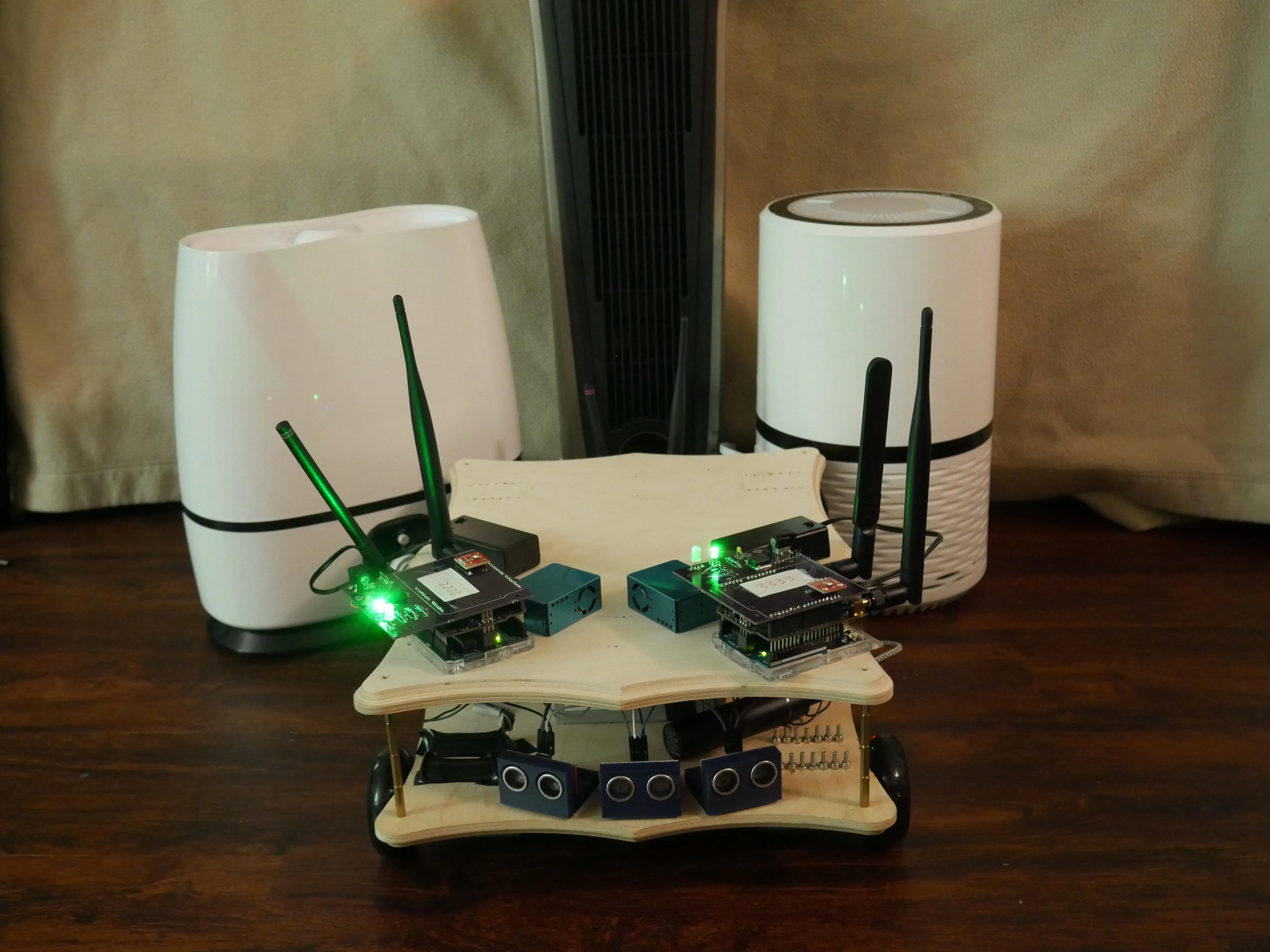AiRobot monitors and modifies the environment in your home by using a robot that receives data from a collection of networked sensors.

• 2,242 views
• 1 comment
• 8 respects

## Components and supplies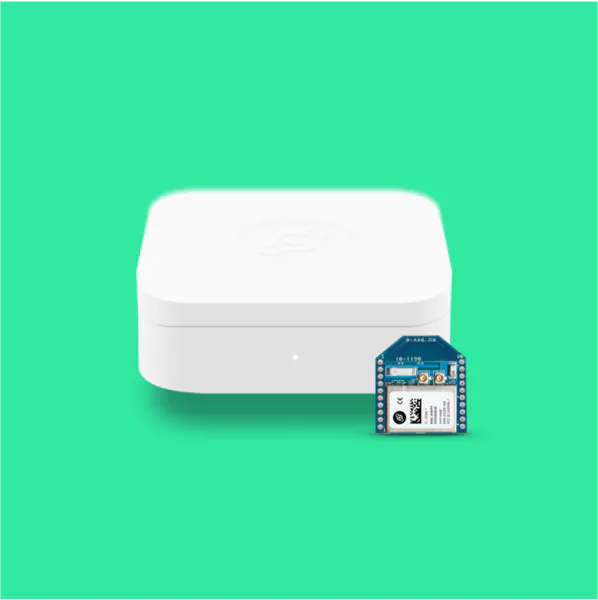Helium Starter Kit (LEGACY) Helium Ethernet kit allows us to connect to the cloud. We used the provided arduino compatible components on the AiRobot
×1
 Helium Arduino/mbed Adapter These Helium Arduino adapters were used on the Arduino Uno's for the AiRobot wall mount sensors
×2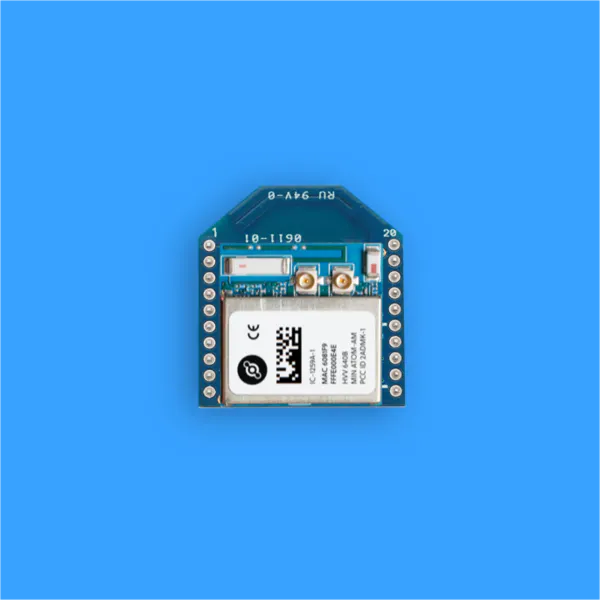Helium Atom Xbee Module These Helium Atom Prototyping Modules were connected to the Helium Arduino Adapters for the AiRobot wall mount sensors
×2Arduino UNO & Genuino UNO The Arduino Uno's were used on the AiRobot wall mount sensors
×2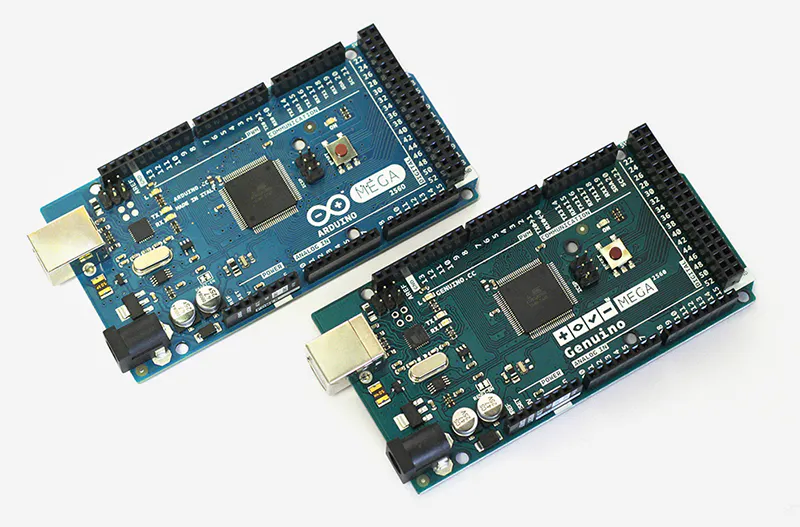Arduino Mega 2560 & Genuino Mega 2560 The Arduino Mega was used on the AiRobot. We needed the vast amount of pins!
×1Raspberry Pi 3 Model B We used the Raspberry Pi on the AiRobot to pull down information from the cloud and talk to the Arduino
×1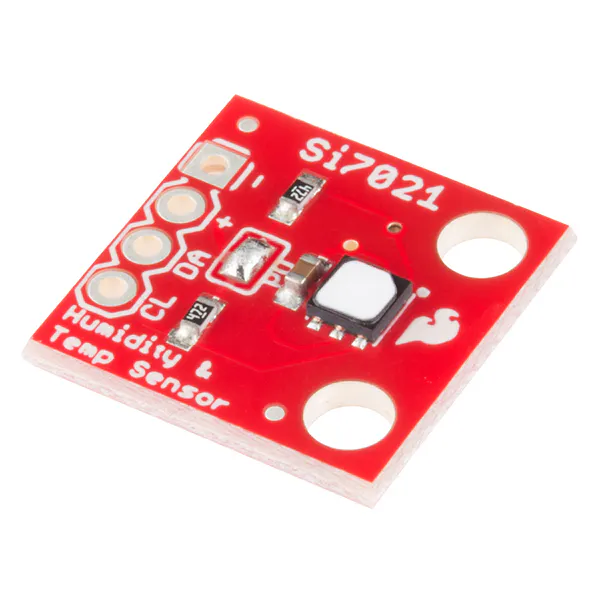SparkFun Humidity and Temperature Sensor Breakout - Si7021 The SparkFun Humidity and Temperature sensors were used on the AiRobot and both AiRobot wall mount sensors!
×3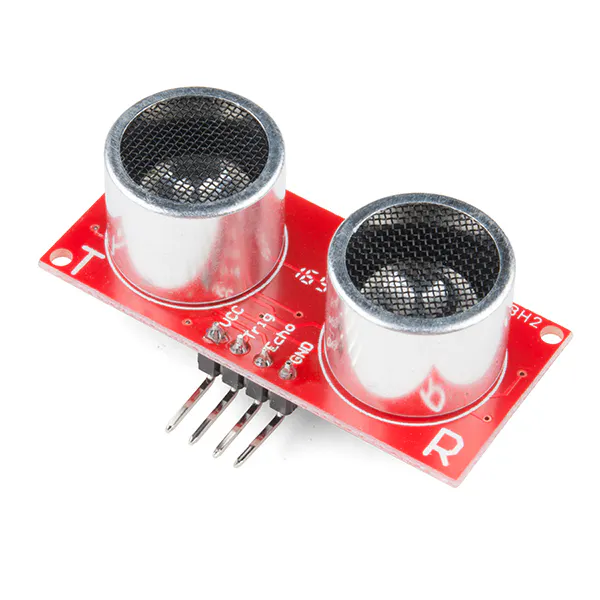SparkFun Ultrasonic Sensor - HC-SR04 3 Ultrasonic Sensors were used on the front of the AiRobot
×3
 PMS5003 - Air Quality Sensor The PMS5003 Air Quality Sensor gives us lots of great data on particle concentration and size distribution! We chose it over the Shinyei Air Quality Sensor for those reasons.
×3
 L298N Motor Drive Controller Board 2 motor drive controllers were used to control all 4 of our motors.
×2
 12V 10.5Ah Car Battery 12V 10.5Ah Lead Acid Battery was a great power system for our robot. We built the system to run for about an hour based on theoretical power consumption calculations!
×1
 Inverter This is an inverter that we used to step up 12V DC to 110V AC. This allows us to power any appliances we put on top of AiRobot.
×1
 Air Purifier We used this air purifier on the AiRobot!
×1
 Humidifier We used this humidifier for the AiRobot testing!
×1
 Lasko Ceramic Tower Heater We used this Ceramic Tower Heater for the AiRobot testing!
×1
 Pre-Cut Wooden Plaque We used these wooden plaques for the AiRobot chassis and the platform for the appliances to sit on.
×2
 Pololu 131:1 Metal Gearmotor We used 4 high torque 12V motors from Pololu. We wanted more torque because the robot might take heavy loads and does not need to move very quickly!
×4
 Pololu Stamped Aluminum L-Bracket L-Bracket used to secure motors onto wooden frame
×4
 Pololu Scooter/Skate Wheel 70×25mm These are the wheels we chose for our robot. We chose them mainly because we wanted to support heavier loads and we knew that we would be testing on hardwood floors. if you're interested in building this robot, and want it to perform better on different terrains, change the wheel size and type.
×4
 Pololu Aluminum Scooter Wheel Adapter This is used to attach the wheel to the motor!
×4
 M3 Standoffs We used M3 Standoffs to separate the chassis from the appliance platform on the AiRobot!
×1
 Zip Ties Zip Ties were used to secure objects and wires onto the frame.
×1Jumper wires (generic) **We used Male to Male, Male to Female, and Female to Female jumper wires!**
×1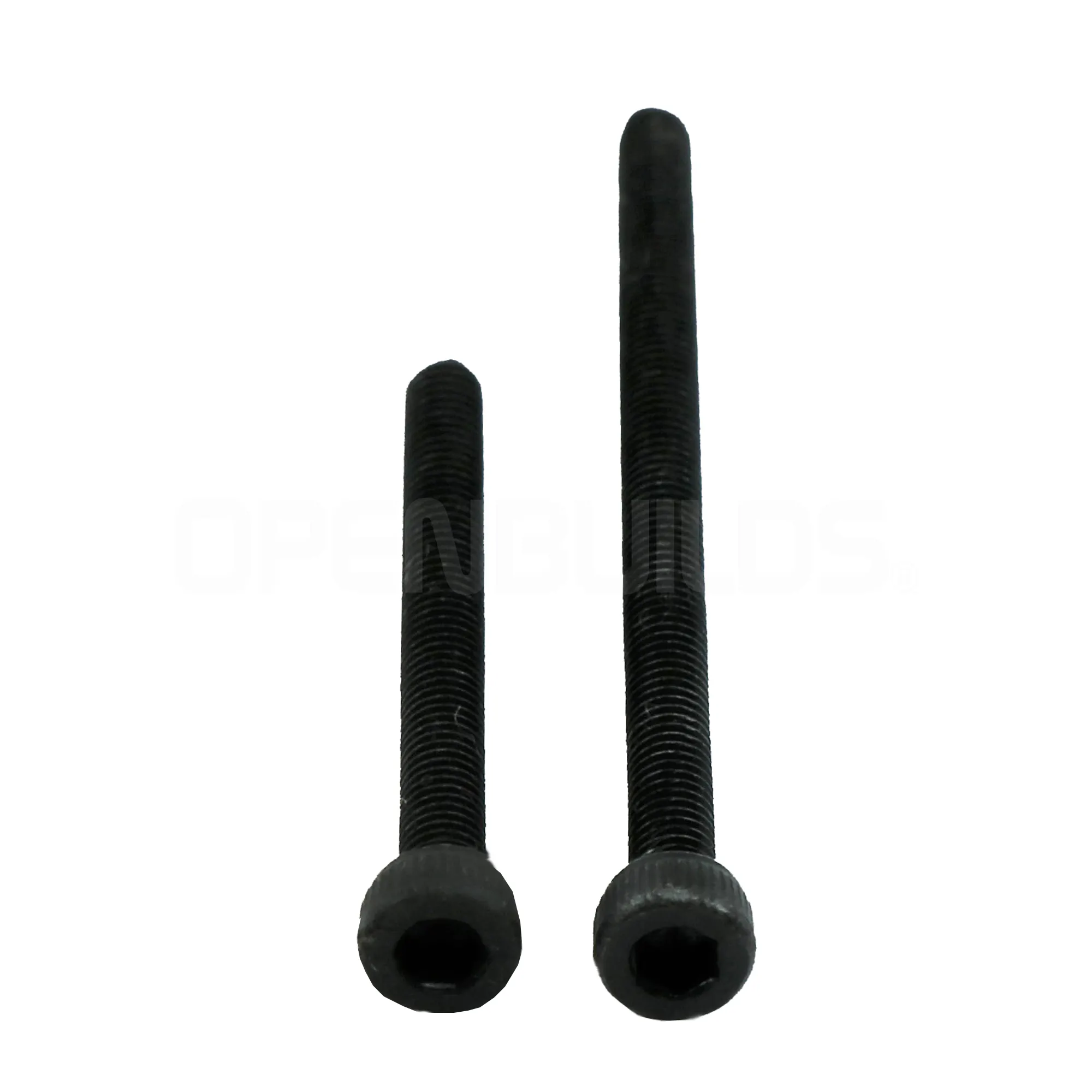OpenBuilds M3 Cap Head Screws Lots and Lots of M3 Screws!
×1OSH Park Custom fabricated PCB We used a custom PCB so we did not have to worry about wires going everywhere! Note that when you buy 1 batch from OSH Park, you get 3 PCB's! The Custom Made PCB Information is available in our project.
×2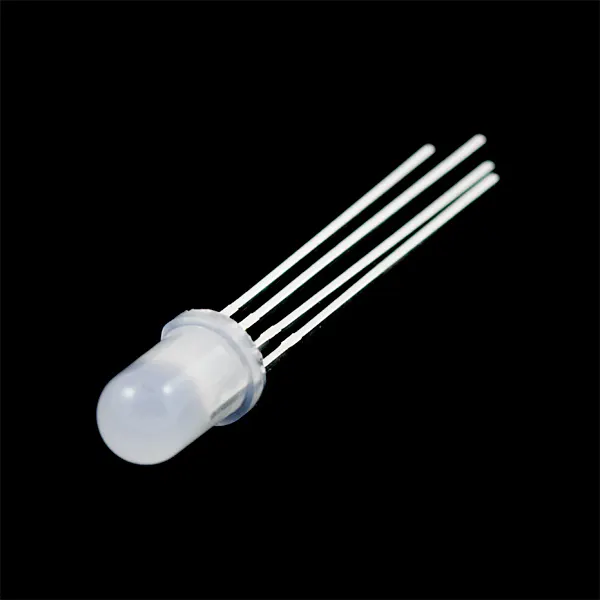RGB Diffused Common Cathode RGB LED is used on the custom PCB for indications
×2
 Green LED Green LED is used to indicate if the board is on or not
×2
 L7805 - 5V Regulator This was used to step down the 9V power for the AiRobot wall mount sensors
×2
 270 Ohm Resistor Resistors used on the custom PCB for the RGB LED and Power Indicator LED
×8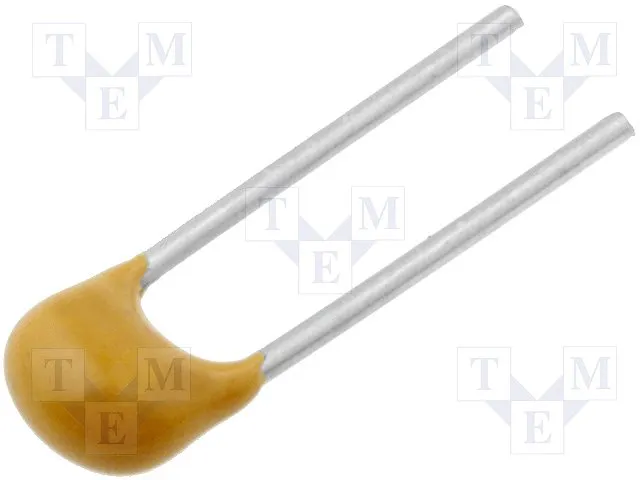Capacitor 100 nF Used to clean the 5V output signal for the L7805 on the AiRobot wall mount sensors
×2
 0.33 uf Capacitor Used to clean the 9V input for the L7805 on the AiRobot wall mount sensors
×2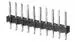Male Header 40 Position 1 Row (0.1") LOTS of header pins for the 2 Arduino Uno's on the AiRobot wall mount sensor pcb's!
×5
 Barrel Jack Connector (Female, PCB) Barrel Jack connector is used to connect the Battery Packs to the AiRobot wall mount sensors
×2
 9v Battery Holder The 9V Battery Holder is used to power the AiRobot wall mount sensor PCBs
×2
 12V 30A SPDT Switch or better
×1
 Barrel Jack (Female) w/ red+black stripped wires
×1
 Terminal Block to Barrel Jack (Male)
×1

## Necessary tools and machines

 Power DrillSoldering iron (generic)3D Printer (generic) 3D Printer is used to print the mounts and cases for: 2x Wallmount Sensor,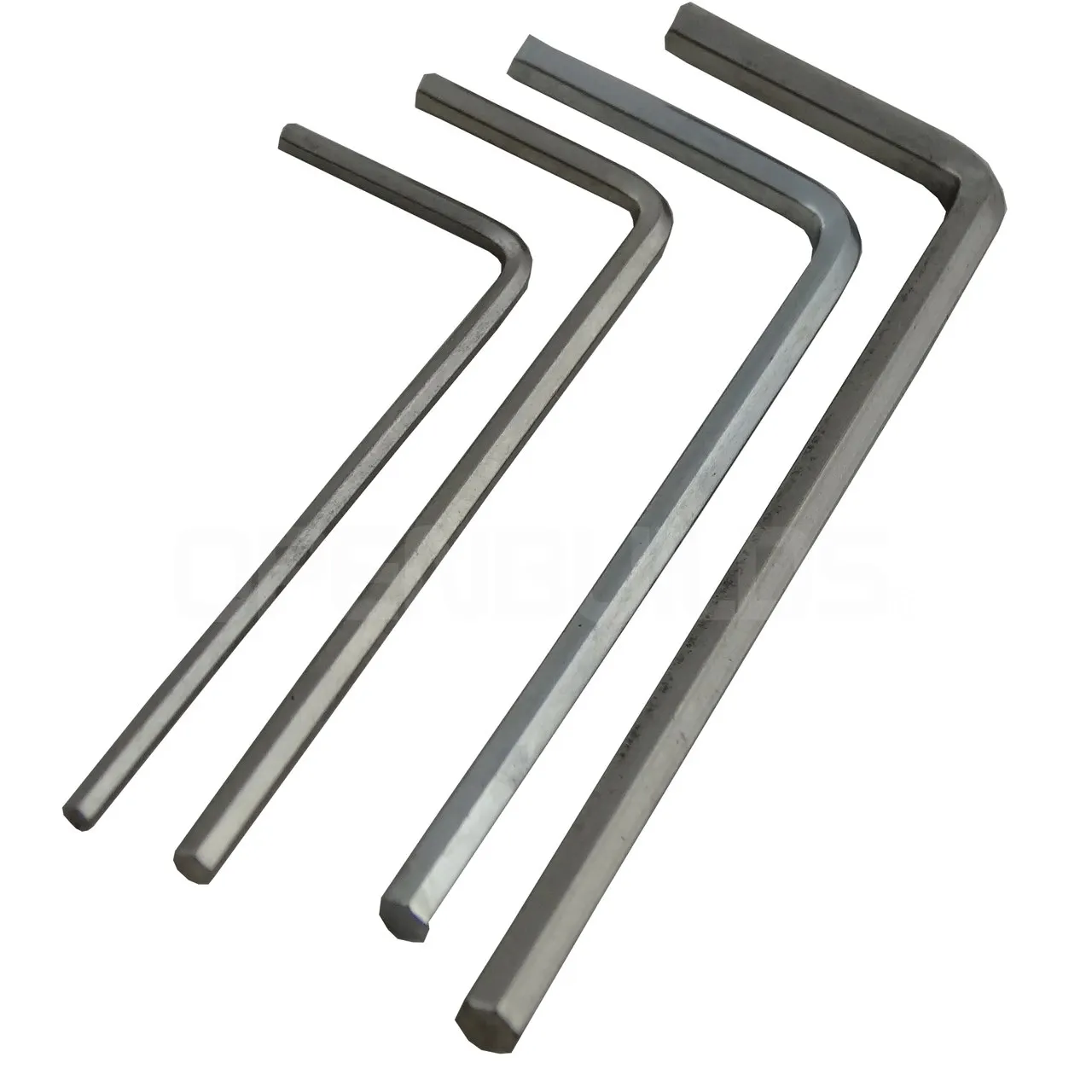OpenBuilds Allen Wrench
 Wire Stripper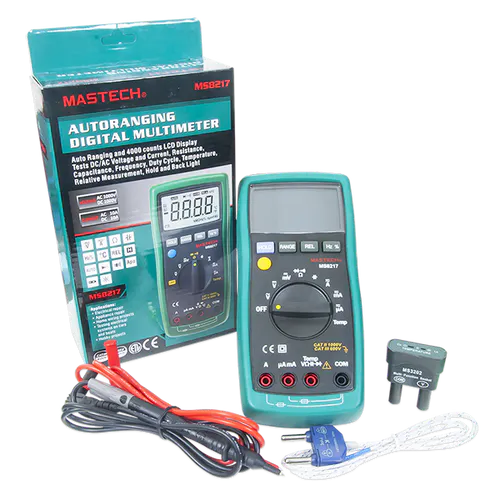Digilent Mastech MS8217 Autorange Digital Multimeter

## Apps and online services

 Raspbian
 Python
 PySerial - Python communication library
 matplotlib (Python)
 numpy (Python)Arduino IDEHelium Dashboard
 DipTrace - PCB editing software

### Overview on the AiRobot System

The AiRobot system comprises of a group of networked sensors and a robot. Every device has sensors that deliver temperature, relative humidity, and air quality measurements to the Google IoT Cloud by using the Helium platform.

The AiRobot is meant for indoor use in homes. AiRobot support sensors are placed a known distance apart and the robot is placed in a calibrated spot. Once the AiRobot is switched on, it will wander between the AiRobot Support Sensors to collect data. The AiRobot knows where it is by using it's motor encoders.

### AiRobot Support Sensors

The AiRobot Support Sensors continuously send temperature, humidity, and air quality information to the Google IoT Cloud through Helium. These Support Sensors are fixed at a known location in a room. This is important to building the several heatmaps.

### AiRobot Support Sensor Schematic

The AiRobot Sensor schematic above gives insight on its capabilities. The Sensor has LEDs to help notify the user about programming and power activity. The Plantower (PMS5003) is our air quality sensor and the Si7021 is the RH/Temp sensor. J3 is a barrel jack connector, U3 are power input pins, and both are connected to U11 which is the L7805, which has an voltage input range of 7V-35V.

Note: we designed the board for a different SparkFun RH/temp sensor than what the board above was built for. It was an easy wiring fix.

### How We Mounted AiRobot Support Sensor

If you look closely on the bottom of the Arduino, we utilize the case with M3 screws to mount. We did not have enough time to 3D print an enclosure, so this was a quick, great, option!

### AiRobot Support Sensor Output & Cloud Data

The photo above shows a test example on how data is sent to the cloud. The AiRobot Support Sensor are the lines that start with 2222.

The data is formatted in the following fashion:

SensorName, Temperature, Relative Humidity, PM0.3um, PM0.5um, PM10um, PM25um, PM50um, PM100um, PM1, PM2.5, PM10, nothing, nothing

What's with all those PM's?

PM stands for particulate matter. The PM03um, PM0.5um, PM35um, PM50um, PM100um are particle size ranges per 0.1L air.

PM1, PM2.5, PM10 are measurements of particle concentration measured in standard units (ug/m3).

### AiRobot

Overview

Below is a picture of an overview of the electronics on AiRobot There is alot of stuff going on, right!?

For AiRobot we used an Arduino Mega for data acquisition and movement, Helium for sending data to the google cloud, and the Raspberry Pi to send serial commands to the robot from the google cloud. The picture below shows that process.

AiRobotPower Structure

### AiRobot Wiring Guide

Here's an interior look at the AiRobot. There is so much stuff going on! We did not have enough time to create a custom PCB to avoid wires traveling everywhere, so we used tape, zip ties, and and whatever we could to keep cables from becoming loose.

Motors

UltrasonicSensors

Temperature,RelativeHumidity,AirQualitySensors

### AiRobot Hardware

Mountingand Securing

To mount objects to the chassis, we used several different methods: Tape, Screws, standoffs, 3D prints, and zip ties.

Standoffs

Standoffs were used to sandwich the two wooden plates together and secure objects on the platform (as seen in the photo above). We also used standoffs to mount the motor controllers to the AiRobot Chassis

ZipTies,3D Prints,Screws

We used zip ties to secure the 12V battery to the AiRobot chassis. The 3D printed parts were used to mount the Ultrasonic Sensors and Temperature/Humidity (SparkFun Si7021) to the chassis. The 3D prints had holes for M3 screws to go through, which were secured using a nut on the opposite side.

DoubleSidedTape

We used double sided tape to mount the PMS5003 (Air Quality) sensor, the Inverter, and the Raspberry Pi.

ArduinoMega+ HeliumMounting

The helium plugs into the Arduino through the pins, which is how it is typically mounted. The Arduino Mega was secured with M3 screws that go through pre drilled through holes on the PCB and case

MotorMounting

We mounted the motors by pencil marking the L bracket hole pattern on the robot and drilling holes through. It was secured using a nut.

AiRobot Drive Train

AiRobot was built with 4 wheel drive. We chose 4 wheel drive because we knew that AiRobot would be carrying a heavy payload (>8kg).

All motors are the same model and have the following specs:

Gear Ratio: 131:1

MaxRPM: 80 RPM

MaxTorque: 250 oz-in

Stall Current: 5 A

The wheels are 75mm Polyurethane scooter wheels from Pololu. The wheel diameter is small because we wanted to support heavy loads. Scooter wheels works best on hard wood floors and has a disadvantage on carpet.

### Battery Considerations

Knowing the power consumption of all the electrical devices we intended to use was important before we purchased a battery. Before building the robot, we created a Power Consumption Table to figure out what kind of 12V battery Ah we needed. To do these calculations, we just used the power equation: P = IV

### Helium IoT Core

In this project we used the Helium IoT Core to get all of our data up to the cloud. The board was easy to use, and worked great! We won't go be creating a tutorial in this section on how to setup the helium to the google cloud, because they already have fantastic documentation on setup!

Here's the helium setup link: https://www.helium.com/dev/hardware-libraries/arduino

We used 3 Helium Atoms with an Arduino adapter to send to 1 Helium channel. All the data goes to 1 file in 1 bucket every 5 minutes on the google cloud.

The data was added every 5 minutes to our bucket using a dataflow. The Google Cloud platform was used to create a virtual machine to help process our data. This was immensely helpful and allowed everything to be all in one place without being cluttered.

Once the data was uploaded to our bucket we used our virtual machine to process it and create heatmaps. Google Cloud Services has excellent tutorials on how to set up everything from virtual machines to Dataflow jobs and I recommend checking their documentation if you experience difficulty. The link to their tutorials page can be found below:

### Heatmap Calculations

After the data was processed and the encoder information was transformed into displacement we needed to calculate the from a few individual points. To create the heatmaps from a bunch of discrete values we used an iterative approach which can be seen in the python code snippet below (for all code see bottom of page).

Each time through the while loop the unfinished heatmaps for temperature, humidity, and particles are copied. We search through every point in the heatmap array and if there is no value provided either by the robot or the supporting sensors we average the cell's 8 neighbors and set it to that value. If it has no neighbors we ensure to stay inside the while loop. After many iterations the discrete values will be expanded based on averaging and a distribution is created.

### Initial Heatmap Testing

In the heatmap graphs below, the origin of the AiRobot is (0, 0). The entire filled area is the bounds of our defined world. The robot starts of traveling in the positive x direction for 4 units (feet). At location [4, 0] the robot turns 90 degrees and travels to [4, -7]. The robot then finishes the rectangle traveling to [0, -7] and back to [0, 0] in that order. Below are the heatmaps for particles, temperature, and humidity for a sample run.

### Heatmap Verification Testing

We wanted to make sure that our heatmap values made sense, so we placed a vaporizer, heater, and air purifier at different known locations in the room.

** In this picture, the AiRobot has sensors on the left side.

**For this testing we used the sensors at the same height as the robot.

The results of the test were promising and showed our robot was working. The following 3 images are the heatmaps for temperature, particles, and humidity. NOTE: THEY ARE ROTATED 90 DEGREES FROM ABOVE IMAGE

The heater is located in the top right corner of this image which lines up well with the region of white denoting a high temperature value.

The humidifier is located in the bottom right corner of this image which is consistent with our results. We see higher number of particles located in that bottom right corner.

The humidity heat map also agrees with our expected results as the humidifier is located in the bottom right corner. There appears to be roughly uniform humidity for the bottom two thirds of the image.

These three images give us stock in AiRobots ability to measure temperature, humidity, and particle distributions through a room.

### Driving to Pollution Source/Region of Interest

With an accurate heatmap the next step is to locate the region of interest and navigate there with the desired appliance. For example, if a region with high concentration of particles was detected an air purifier could be mounted on AiRobot and it would drive to the source. By driving to the source AiRobot is best able to improve air quality or issues. Appliances we used were a humidifier and air purifier. To navigate AiRobot to a spot on the heatmap the distance from Airobot's currently location and the bad spot was calculated. This was calculated on GCS and a txt file of the desired navigation commands was outputted. Our RPi then transferred the commands through serial and directed the robot to its desired location.

### Programming Instructions

Due to the massive amount of information, we struggled providing a step by step tutorial on how to fully build all components of AiRobot. But in this section, we hope to provide you some insight on how the programming works! All of our code is attached to this project as well.

The generic structure is laid out as follows:

1) Arduino Mega wanders around room reading encoders, particles sensor, temperature sensor, and humidity sensor. All this data is continuously streamed to the Google Cloud using helium.

2) After enough data is sent to Google cloud our virtual machine processes encoder information and converts it distance. Heatmaps are created using these distance measurements and the sensor values (temperature, humidity, particle concentration).

3) Point of interest is calculated from the heatmaps and serial directions on how to navigate there are calculated from virtual machine and uploaded to Google Cloud.

4) RPi copies directions from Google Cloud and transmits it to arduino through serial. This directs AiRobot to its final position where it stays and runs the desired appliance.

5) Lastly code runs on the virtual machine tracks sensor measurements in AiRobots location to monitor progress of cleaning the polluted area.

### Obstacles we encountered

This project was not easy. We encountered obstacles all along the way. Thanks to all nighters and never giving up, we overcame them.

Some problems we encountered were:

1. Time. This project was an enormous amount of work that was done in a short period of time. We are only a team of 2!

2.Sleep. Towards the submission date, we pulled several all nighters to work hard and overcome all obstacles we had to.

3. PartSelection. Given the time constraints and the complexity of this project, we were forced to make quick decisions about parts

3.a Motor Selection. When choosing the motors to use, we had to theoretically make sure that it was in the spec to carry our intended load. This load is based on alot of things, since we have alot of parts.

4.PowerConsumption. Depending on the appliance you wanted to put on the AiRobot platform, the weight of the unit and the total power consumption increases. When the power consumption increases, it changes our required lead acid battery size, which means much larger % changes in weight. Lead acid batteries are HEAVY!

5.Robot Positioning. We tried GPS - it was not good enough. We tried an accelerometer - it was not good enough. Signal Strength was not good enough. We had too little time to learn IR pattern recognition with a camera. This was an extremely tough obstacle, so we just relied on encoders.

6.Encoders. We had trouble reading all values of the encoders at the same time. This was a very annoying problem. We were forced to rely on encoder data from one wheel.

7. Monitoringand controllingSOMANY THINGS. Very stressful, very difficult. So much code that needed to be written.

### Possible Future Improvements to AiRobot

We didn't have alot of time on this project, so we could not make AiRobot perfect. We're very satisfied with the work we completed though! Some future improvements:

1.Moreaccurate Positioning. We believe that this is the hardest part of this entire project. We believe that the IR method that Broomba's use might by the best chance to get this to work.

2.Wander mode. Once we have more accurate positioning, AiRobot can start from anywhere without being calibrated. It can truely walk randomly within a room.

3.AiRobotAppliances. If we were to hack or build the appliances we put on the AiRobot platform, we could customly modify the environment based on the data we receive without having to touch a button.

### Robot Code:

``//Libraries#include "SparkFun_Si7021_Breakout_Library.h"#include <Wire.h>#include <Helium.h>#include <SoftwareSerial.h>#include "Arduino.h"#include "Board.h"#include "Helium.h"#include "HeliumUtil.h"//Definitions - Encoder A and B//Code for pin names//First digit: R,L for right and left//Second digit: F,B for front and back//Third digit: A,B for encoder A and encoder B#define RFA 18#define RFB 19#define RBA 50#define RBB 51#define LFA 2 //LFA PIN CHANGED FROM 42 to 2#define LFB 3 //LFB PIN CHANGED FROM 43 TO 3#define LBA 48#define LBB 49//Definitions - Enable Pins#define RFE 11 //RFE PIN CHANGED from 2 TO 11#define RBE 9 //RBE PIN CHANGED FROM 3 to 9#define LFE 4#define LBE 5//Definitions - Motor Poles#define RF1 26#define RF2 27#define RB1 28#define RB2 29#define LF1 7#define LF2 8#define LB1 32#define LB2 33//Definitions - Encodersint RFval;int RBval;int LFval;int LBval;int RFpos = 0;int RBpos = 0;int LFpos = 0;int LBpos = 0;int RFlast = LOW;int RBlast = LOW;int LFlast = LOW;int LBlast = LOW;int RFn = LOW;int RBn = LOW;int LFn = LOW;int LBn = LOW;// wheel information definitionsconst int diameter = 70; // in mmconst int pi = 3.141592654;const int circumference = diameter * pi;const int tickspercm = 360 / (diameter*pi);//---------------------------------------//Setup Serial Communication intString Direction;           //the direction that the roobt will drive inString distance;               //the distance you want to travelString angle;//Ultrasonic Sensorsconst int trigPin1 = 36;const int echoPin1 = 37;const int trigPin2 = 38;const int echoPin2 = 39;const int trigPin3 = 40;const int echoPin3 = 41;// defines variableslong duration1;int distance1;long duration2;int distance2;long duration3;int distance3;int Boundary = 20;bool first_on = true;int RF_pos_copy = 0;int TURNS = 0;//Setup Software Serial for SensorsSoftwareSerial pmsSerial(10, 11);Weather sensor;//Set initial Float Valuesfloat humidity = 0;float tempf = 0;//Helium ChannelHelium  helium(&atom_serial);Channel channel(&helium);void report_status(int status){   if (helium_status_OK == status)   {       Serial.println("Succeeded");   }   else   {       Serial.println("Failed");   }}void report_status_result(int status, int result){   if (helium_status_OK == status)   {       if (result == 0)       {           Serial.println("Succeeded");       }       else {           Serial.print("Failed - ");           Serial.println(result);       }   }   else   {       Serial.println("Failed");   }}void setup() { Serial.begin(9600); //HELIUM Initialization // Begin communication with the Helium Atom   // The baud rate differs per supported board   // and is configured in Board.h   helium.begin(HELIUM_BAUD_RATE);   // Connect the Atom to the Helium Network   Serial.print("Connecting - ");   int status = helium.connect();   int8_t result;   Serial.print("Creating Channel - ");   status = channel.begin("env-hub", &result);   // Print status and result   report_status_result(status, result);   //PMS5003 Baud Rate is 9600   pmsSerial.begin(9600); //Initialize the Si7021 and ping it   sensor.begin(); //Set Outputs pinMode(RFA, INPUT); pinMode(RFB, INPUT); pinMode(RBA, INPUT); pinMode(RBB, INPUT); pinMode(LFA, INPUT); pinMode(LFB, INPUT); pinMode(LBA, INPUT); pinMode(LBB, INPUT); pinMode(RFE, OUTPUT); pinMode(RBE, OUTPUT); pinMode(LFE, OUTPUT); pinMode(LBE, OUTPUT); pinMode(RF1, OUTPUT); pinMode(RF2, OUTPUT); pinMode(RB1, OUTPUT); pinMode(RB2, OUTPUT); pinMode(LF1, OUTPUT); pinMode(LF2, OUTPUT); pinMode(LB1, OUTPUT); pinMode(LB2, OUTPUT); pinMode(trigPin1, OUTPUT); // Sets the trigPin as an Output pinMode(echoPin1, INPUT); // Sets the echoPin as an Input pinMode(trigPin2, OUTPUT); // Sets the trigPin as an Output pinMode(echoPin2, INPUT); // Sets the echoPin as an Input pinMode(trigPin3, OUTPUT); // Sets the trigPin as an Output pinMode(echoPin3, INPUT); // Sets the echoPin as an Input //Motor Related Initilizations digitalWrite(RFA, LOW); digitalWrite(RFB, LOW); digitalWrite(RBA, LOW); digitalWrite(RBB, LOW); digitalWrite(LFA, LOW); digitalWrite(LFB, LOW); digitalWrite(LBA, LOW); digitalWrite(LBB, LOW); digitalWrite(RFE, LOW); digitalWrite(RBE, LOW); digitalWrite(LFE, LOW); digitalWrite(LBE, LOW); digitalWrite(RF1, LOW); digitalWrite(RF2, LOW); digitalWrite(RB1, LOW); digitalWrite(RB2, LOW); digitalWrite(LF1, LOW); digitalWrite(LF2, LOW); digitalWrite(LB1, LOW); digitalWrite(LB2, LOW); //Initialize the Si7021 //sensor.begin(); //WE CHANGED THIS AND WE NEED TO GET IT RUNNING}struct pms5003data { uint16_t framelen; uint16_t pm10_standard, pm25_standard, pm100_standard; uint16_t pm10_env, pm25_env, pm100_env; uint16_t particles_03um, particles_05um, particles_10um, particles_25um, particles_50um, particles_100um; uint16_t unused; uint16_t checksum;};struct pms5003data data;void loop() { if (Serial.available() > 0)                         //if the user has sent a command {   Direction = Serial.readStringUntil(' ');       //read the characters in the command until you reach the first space   distance = Serial.readStringUntil(' ');           //read the characters in the command until you reach the second space   angle = Serial.readStringUntil(' ');   //print the command that was just received in the serial monitor   Serial.print(Direction);   Serial.print(" ");   Serial.println(distance.toInt());   if (Direction == "m")                         //if the entered direction is forward   {     Move(distance.toInt());   }   if (Direction == "t")                         //if the entered direction is forward    {     Turn(distance.toInt());   }   if (Direction == "r")                         //if the entered direction is forward   {     if (distance.toInt()==0){       //monitor serial for movement commands       //once at desired location then run r 1,2,3 depending on desired sensor       Serial.println("waiting for commands")       while (true) {           if (Serial.available() > 0)                         //if the user has sent a command {   Direction = Serial.readStringUntil(' ');       //read the characters in the command until you reach the first space   distance = Serial.readStringUntil(' ');           //read the characters in the command until you reach the second space   angle = Serial.readStringUntil(' ');   //print the command that was just received in the serial monitor   Serial.print(Direction);   Serial.print(" ");   Serial.println(distance.toInt());   if (Direction == "m")                         //if the entered direction is forward   {     Move(distance.toInt());   }   if (Direction == "t")                         //if the entered direction is forward    {     Turn(distance.toInt());   }   if (Direction == "r")                         //if the entered direction is forward   {     if (distance.toInt()>0){   if (distance.toInt()==1){       char buffer;       while (true){         tempf = sensor.getTempF();         String T = dtostrf(tempf, 0, 2, buffer);           int8_t result;           String HeliumData="1111,"+ T;           const char * Data = HeliumData.c_str();           Serial.println(Data); //Test Diagnostic           int status = channel.send(Data, strlen(Data), &result);         }     }     if (distance.toInt()==2){       char buffer;       while (true){         humidity = sensor.getRH();         String H = dtostrf(humidity, 0, 2, buffer);           int8_t result;           String HeliumData="1111,"+ H;           const char * Data = HeliumData.c_str();           Serial.println(Data); //Test Diagnostic           int status = channel.send(Data, strlen(Data), &result);         }     }     if (distance.toInt()==3){       char buffer;       while (true){         if (readPMSdata(&pmsSerial)) {  /*        // Size ranges of particles - Good for histogram plot // Particles > 0.3um / 0.1L air String PM_03um = dtostrf(data.particles_03um, 0, 0, buffer); //Particles > 0.5um / 0.1L air String PM_05um = dtostrf(data.particles_05um, 0, 0, buffer); //Particles > 1.0um / 0.1L air String PM_10um = dtostrf(data.particles_10um, 0, 0, buffer); //Particles > 2.5um / 0.1L air String PM_25um = dtostrf(data.particles_25um, 0, 0, buffer); //Particles > 5.0um / 0.1L air String PM_50um = dtostrf(data.particles_50um, 0, 0, buffer); //Particles > 50 um / 0.1L air String PM_100um = dtostrf(data.particles_100um, 0, 0, buffer); */ // Concentration Units (standard) // PM 1.0 //String PM1 = dtostrf(data.pm10_standard, 0, 0, buffer); // PM 2.5 String PM2p5 = dtostrf(data.pm25_standard, 0, 0, buffer); // PM 10 //String PM10 = dtostrf(data.pm100_standard, 0, 0, buffer);           int8_t result;           String HeliumData="1111,"+ PM2p5;           const char * Data = HeliumData.c_str();           Serial.println(Data); //Test Diagnostic           int status = channel.send(Data, strlen(Data), &result);         }         }     }   }         }     }     }     } } } else {   //blah                               //turn the motors } if (readPMSdata(&pmsSerial)) {   humidity = sensor.getRH();   tempf = sensor.getTempF();//----------------------------------------------------------// String Section String SensorName = "1111"; //2222,3333,etc. char buffer; // Size ranges of particles - Good for histogram plot // Particles > 0.3um / 0.1L air String PM_03um = dtostrf(data.particles_03um, 0, 0, buffer); //Particles > 0.5um / 0.1L air String PM_05um = dtostrf(data.particles_05um, 0, 0, buffer); //Particles > 1.0um / 0.1L air String PM_10um = dtostrf(data.particles_10um, 0, 0, buffer); //Particles > 2.5um / 0.1L air String PM_25um = dtostrf(data.particles_25um, 0, 0, buffer); //Particles > 5.0um / 0.1L air String PM_50um = dtostrf(data.particles_50um, 0, 0, buffer); //Particles > 50 um / 0.1L air String PM_100um = dtostrf(data.particles_100um, 0, 0, buffer); // Concentration Units (standard) // PM 1.0 String PM1 = dtostrf(data.pm10_standard, 0, 0, buffer); // PM 2.5 String PM2p5 = dtostrf(data.pm25_standard, 0, 0, buffer); // PM 10 String PM10 = dtostrf(data.pm100_standard, 0, 0, buffer); //Temperature RH String Tempf = dtostrf(tempf, 0, 2, buffer); String Humidity = dtostrf(humidity, 0, 2, buffer); //Convert all strings into One String to rule them all. **********USE THIS STRING TO SEND TO HELIUM!*********** int8_t result; if (first_on==true){   first_on=false;   String HeliumData="1111,-9999,0,0,0,0,0,0,0,0,0,0,0";   const char * Data = HeliumData.c_str();   Serial.println(Data); //Test Diagnostic   delay(1);   int status = channel.send(Data, strlen(Data), &result);   } String HeliumData = SensorName + "," + Tempf + "," + Humidity + PM_03um + "," + PM_05um + "," + PM_10um + "," + PM_25um + "," + PM_50um + "," + PM_100um + "," + PM1 + "," + PM2p5 + "," + PM10 + ","+String(RF_pos_copy)+","+String(TURNS); const char * Data = HeliumData.c_str();  Serial.println(Data); //Test Diagnostic Serial.println(RF_pos_copy); Serial.println(TURNS); //after turns gets uploaded set back to 0 TURNS=0; // Send data to channel //const char * SendData = HeliumData; int status = channel.send(Data, strlen(Data), &result); //report_status_result(status, result); }}boolean readPMSdata(Stream *s) { if (! s->available()) {   return false; } // Read a byte at a time until we get the'0x42' start-byte if (s->peek() != 0x42) {   s->read();   return false; } if (s->available() < 32) {   return false; } uint8_t buffer;     uint16_t sum = 0; s->readBytes(buffer, 32); // get checksum ready for (uint8_t i=0; i<30; i++) {   sum += buffer[i]; } uint16_t buffer_u16; for (uint8_t i=0; i<15; i++) {   buffer_u16[i] = buffer[2 + i*2 + 1];   buffer_u16[i] += (buffer[2 + i*2] << 8); } memcpy((void *)&data, (void *)buffer_u16, 30); if (sum != data.checksum) {   //Serial.println("Checksum failure");   return false; } return true;}//void Move(int distance)                       //function for driving the right motor{ digitalWrite(RFE, HIGH); digitalWrite(RBE, HIGH); digitalWrite(LFE, HIGH); digitalWrite(LBE, HIGH); if (distance > 0) {   while (RFpos < distance) {     RFn = digitalRead(RFA);     if ((RFlast == LOW) && (RFn == HIGH)) {       if (digitalRead(RFB) == LOW) {         RFpos--;         RF_pos_copy--;       }       else {         RFpos++;         RF_pos_copy++;       }       if((distance2<Boundary and distance2>0) or (distance1<Boundary and distance1>0) or (distance3<Boundary and distance3>0)){ //or distance1<Boundary or distance3<Boundary         Serial.print(distance2);         Serial.println(",OBJECT!");         RFpos=0;         distance2=0;         Stop();         break;       }       Serial.println(RFpos);       Serial.println(RF_pos_copy);//        Serial.print(",");//        Serial.print(distance1);//        Serial.print(",");//        Serial.print(distance2);//        Serial.print(",");//        Serial.println(distance3);     }     RFlast = RFn;     digitalWrite(RF1, HIGH);     digitalWrite(RB2, HIGH);     digitalWrite(LF1, HIGH);     digitalWrite(LB2, HIGH);     digitalWrite(LF2, LOW);     digitalWrite(LB1, LOW);     digitalWrite(RF2, LOW);     digitalWrite(RB1, LOW);   }   RFpos = 0;   Stop(); } if (distance < 0) {//    analogWrite(RFE, 215); analogWrite(RBE, 215);//    analogWrite(LFE, 215); analogWrite(LBE, 215);   while (RFpos > distance) {     RFn = digitalRead(RFA);     if ((RFlast == LOW) && (RFn == HIGH)) {       if (digitalRead(RFB) == LOW) {         RFpos--;         RF_pos_copy--;       }       else {         RFpos++;         RF_pos_copy++;       }       Serial.println(RFpos);     }     RFlast = RFn;         digitalWrite(RF1, LOW);         digitalWrite(RB2, LOW);         digitalWrite(LF1, LOW);         digitalWrite(LB2, LOW);         digitalWrite(LF2, HIGH);         digitalWrite(LB1, HIGH);         digitalWrite(RF2, HIGH);         digitalWrite(RB1, HIGH);   } RFpos = 0; Stop(); }}void Turn(int angle) { digitalWrite(RFE, HIGH); digitalWrite(RBE, HIGH); digitalWrite(LFE, HIGH); digitalWrite(LBE, HIGH); if (angle > 0) {   while (RFpos < angle) {     RFn = digitalRead(RFA);     if ((RFlast == LOW) && (RFn == HIGH)) {       if (digitalRead(RFB) == LOW) {         RFpos--;       }       else {         RFpos++;       }       Serial.println(RFpos);       //Serial.print(",");     }     RFlast = RFn;     digitalWrite(RF2, LOW);     digitalWrite(RB1, LOW);     digitalWrite(LF1, LOW);     digitalWrite(LB2, LOW);     digitalWrite(RF1, HIGH);     digitalWrite(RB2, HIGH);     digitalWrite(LF2, HIGH);     digitalWrite(LB1, HIGH);   }         if (RFpos>90){         TURNS=1;}         if (RFpos<-90) {TURNS=-1;}   RFpos = 0;   Stop(); } if (angle < 0) {   while (RFpos > angle) {     RFn = digitalRead(RFA);     if ((RFlast == LOW) && (RFn == HIGH)) {       if (digitalRead(RFB) == LOW) {         RFpos--;       }       else {         RFpos++;       }       Serial.println(RFpos);     }     RFlast = RFn;     digitalWrite(RF1, LOW);     digitalWrite(RB2, LOW);     digitalWrite(LF2, LOW);     digitalWrite(LB1, LOW);     digitalWrite(RF2, HIGH);     digitalWrite(RB1, HIGH);     digitalWrite(LF1, HIGH);     digitalWrite(LB2, HIGH);   }         if (RFpos>90){         TURNS=1;}         if  (RFpos<-90){TURNS=-1;}   RFpos = 0;   Stop(); }}void Stop() { digitalWrite(LF2, LOW); digitalWrite(LB1, LOW); digitalWrite(RF2, LOW); digitalWrite(RB1, LOW); digitalWrite(RF1, LOW); digitalWrite(RB2, LOW); digitalWrite(LF1, LOW); digitalWrite(LB2, LOW); delay(250);}int Ultrasonic() { // Clears the trigPin digitalWrite(trigPin1, LOW); delayMicroseconds(2); // Sets the trigPin on HIGH state for 10 micro seconds digitalWrite(trigPin1, HIGH); delayMicroseconds(10); digitalWrite(trigPin1, LOW); // Reads the echoPin, returns the sound wave travel time in microseconds duration1 = pulseIn(echoPin1, HIGH); // Calculating the distance distance1 = duration1 * 0.034 / 2; // Prints the distance on the Serial Monitor //Serial.print("Distance1: "); //Serial.print(distance1); //Serial.print(","); delay(10); // Clears the trigPin digitalWrite(trigPin2, LOW); delayMicroseconds(2); // Sets the trigPin on HIGH state for 10 micro seconds digitalWrite(trigPin2, HIGH); delayMicroseconds(10); digitalWrite(trigPin2, LOW); // Reads the echoPin, returns the sound wave travel time in microseconds duration2 = pulseIn(echoPin2, HIGH); // Calculating the distance distance2 = duration2 * 0.034 / 2; // Prints the distance on the Serial Monitor //Serial.print("Distance2: "); //Serial.print(distance2); //Serial.print(","); delay(10); // Clears the trigPin digitalWrite(trigPin3, LOW); delayMicroseconds(2); // Sets the trigPin on HIGH state for 10 micro seconds digitalWrite(trigPin3, HIGH); delayMicroseconds(10); digitalWrite(trigPin3, LOW); // Reads the echoPin, returns the sound wave travel time in microseconds duration3 = pulseIn(echoPin3, HIGH); // Calculating the distance distance3 = duration3 * 0.034 / 2; // Prints the distance on the Serial Monitor //Serial.print("Distance3: "); //Serial.println(distance3); delay(10);}``

### Heatmap Generator

``import numpy as npimport timeimport osimport globimport matplotlibmatplotlib.use('Agg')import matplotlib.pyplot as pltimport matplotlib.path as mpath``

import random

``import copy as cp# Remove all old files from VM instancedel_files=glob.glob("/home/blainejayotte/data_download/files/*")for f in del_files:	os.remove(f)# Copy in new files from GCSos.system("gsutil cp gs://mybuckettest12345/data/* /home/blainejayotte/data_download/files/")#os.system("gsutil cp gs://mybuckettest12345/saved_data/output2018-07-15T14:35:00.000Z-2018-07-15T14:40:00.000Z-pane-0-last-00-of-01 /home/blainejayotte/data_download/files/")os.chdir("/home/blainejayotte/data_download/files/")# Remove all GCS txt files#os.system("gsutil rm gs://mybuckettest12345/data/*")# Read each line of the new fileslines=[]for filename in os.listdir(os.getcwd()):	new_lines=[line.rstrip('\n') for line in open(filename)]	for j in range(0,len(new_lines)):		lines.append(new_lines[j].split(','))all_data=np.zeros((len(lines),13))for i in range(0,len(lines)):	all_data[i]=float(lines[i])	all_data[i]=float(lines[i])	all_data[i]=float(lines[i])	all_data[i]=float(lines[i])	all_data[i]=float(lines[i])	all_data[i]=float(lines[i])	all_data[i]=float(lines[i])	all_data[i]=float(lines[i])	all_data[i]=float(lines[i])	all_data[i]=float(lines[i])	all_data[i]=float(lines[i])	all_data[i]=float(lines[i])	all_data[i]=float(lines[i])# Filter data into S1,S2,S3: Array for each sensorS1=all_data[all_data[:,0] == 1111]S2=all_data[all_data[:,0] == 2222]S3=all_data[all_data[:,0] == 3333]# delete all data before row in S1 with only len=2append=Falsecreate=Trueclean_S1=np.zeros((len(S1),13))ff=0for i in range(ff,len(S1)-2):	if append==True:		if create==True:			strt=i			clean_S1=np.zeros((len(S1)-strt-2,13))			create=False		clean_S1[i-strt]=S1[i]		clean_S1[i-strt]=S1[i]		clean_S1[i-strt]=S1[i]		clean_S1[i-strt]=S1[i]		clean_S1[i-strt]=S1[i]		clean_S1[i-strt]=S1[i]		clean_S1[i-strt]=S1[i]		clean_S1[i-strt]=S1[i]		clean_S1[i-strt]=S1[i]		clean_S1[i-strt]=S1[i]		clean_S1[i-strt]=S1[i]		clean_S1[i-strt]=S1[i]		clean_S1[i-strt]=S1[i]	if S1[i+2]!=0 and append==False:		append=TrueS1_pos=np.zeros((len(clean_S1),2))# need to clean up encoder and turn information and convert to positiontpf=120direction=[1,0]pos=[0,0]for i in range(1,len(clean_S1)):	turn=clean_S1[i]	#update position	pos=pos+direction*(clean_S1[i]-clean_S1[i-1])/tpf	pos=pos+direction*(clean_S1[i]-clean_S1[i-1])/tpf	S1_pos[i]=pos	S1_pos[i]=pos	#update direction	if turn==1: #CCW		if direction==[1,0]:			direction=[0,1]		elif direction==[-1,0]:                       direction=[0,-1]		elif direction==[0,1]:                       direction=[-1,0]		elif direction==[0,-1]:                       direction=[1,0]	if turn==-1: #CW		if direction==[1,0]:                       direction=[0,-1]               elif direction==[-1,0]:                       direction=[0,1]               elif direction==[0,1]:                       direction=[1,0]               elif direction==[0,-1]:                       direction=[-1,0]print(S1_pos)# y,xS2_loc=[3,5]S3_loc=[-9,-1]#Create meshgridmesh_square=1 # in ft# these values need to be rounded to nearest half foot (or foot depends on resolution)S1xmin=int(round(np.amin(S1_pos[:,0])))S1xmax=int(round(np.amax(S1_pos[:,0])))S1ymin=int(round(np.amin(S1_pos[:,1])))S1ymax=int(round(np.amax(S1_pos[:,1])))#determine if sensors are mins or maxs compared to robotxmin=min(S1xmin,S2_loc,S3_loc)xmax=max(S1xmax,S2_loc,S3_loc)ymin=min(S1ymin,S2_loc,S3_loc)ymax=max(S1ymax,S2_loc,S3_loc)x=np.arange(int(xmin/mesh_square)-mesh_square,int(xmax/mesh_square)+mesh_square,mesh_square)y=np.arange(int(ymin/mesh_square)-mesh_square,int(ymax/mesh_square)+mesh_square,mesh_square)xx,yy=np.meshgrid(x,y)w,h=xx.shape# NOTE: heatmap plot cuts off last row and column so ignore itZ_temp=np.zeros((w, h))Z_humid=np.zeros((w, h))Z_particle=np.zeros((w, h))for i in range(0,len(clean_S1)):	Z_temp[int(S1_pos[i]/mesh_square)-ymin][int(S1_pos[i]/mesh_square)-xmin]=clean_S1[i]	Z_humid[int(S1_pos[i]/mesh_square)-ymin][int(S1_pos[i]/mesh_square)-xmin]=clean_S1[i]	Z_particle[int(S1_pos[i]/mesh_square)-ymin][int(S1_pos[i]/mesh_square)-xmin]=clean_S1[i]Z_temp[int(S2_loc/mesh_square)-ymin][int(S2_loc/mesh_square)-xmin]=S2Z_temp[int(S3_loc/mesh_square)-ymin][int(S3_loc/mesh_square)-xmin]=S3Z_humid[int(S2_loc/mesh_square)-ymin][int(S2_loc/mesh_square)-xmin]=S2Z_humid[int(S3_loc/mesh_square)-ymin][int(S3_loc/mesh_square)-xmin]=S3Z_particle[int(S2_loc/mesh_square)-ymin][int(S2_loc/mesh_square)-xmin]=S2Z_particle[int(S3_loc/mesh_square)-ymin][int(S3_loc/mesh_square)-xmin]=S3# Filling in all the values# algorithm goes through all "empty" points and sets equal to average of 8 neighbors or closest valueloop=Truewhile loop==True:	loop=False	Z_copy_temp=cp.deepcopy(Z_temp)	Z_copy_humid=cp.deepcopy(Z_humid)	Z_copy_particle=cp.deepcopy(Z_particle)	for i in range(0,w-1):		for j in range(0,h-1):			if Z_copy_temp[i][j]==0:				#find average of 8 neighbors				avg_temp=0				avg_humid=0				avg_particle=0				avg_cnt=0				rng=1				for row in range(-rng,rng+1):					for col in range(-rng,rng+1):						try:							val=Z_copy_temp[i+row][j+col]							if val!=0:								avg_temp+=Z_copy_temp[i+row][j+col]								avg_humid+=Z_copy_humid[i+row][j+col]								avg_particle+=Z_copy_particle[i+row][j+col]								avg_cnt+=1						except:							pass				if avg_cnt==0:					loop=True				else:					Z_temp[i][j]=avg_temp/avg_cnt					Z_humid[i][j]=avg_humid/avg_cnt					Z_particle[i][j]=avg_particle/avg_cntos.chdir("/home/blainejayotte/data_download/heatmaps/")fig_temp=plt.figure()ax_temp=fig_temp.add_subplot(111)pc_temp=ax_temp.pcolormesh(xx,yy,Z_temp,cmap='hot')fig_temp.colorbar(pc_temp)ax_temp.set_title('Temperature Heatmap')plt.show()timestr = time.strftime("%Y_%m_%d_%H_%M_%S")heatmap_name_temp='heatmap_temp_'+timestrplt.savefig(heatmap_name_temp)fig_humid=plt.figure()ax_humid=fig_humid.add_subplot(111)pc_humid=ax_humid.pcolormesh(xx,yy,Z_humid,cmap='hot')fig_humid.colorbar(pc_humid)ax_humid.set_title('Humidity Heatmap')plt.show()heatmap_name_humid='heatmap_humid_'+timestrplt.savefig(heatmap_name_humid)fig_particle=plt.figure()ax_particle=fig_particle.add_subplot(111)pc_particle=ax_particle.pcolormesh(xx,yy,Z_particle,cmap='hot')fig_particle.colorbar(pc_particle)ax_particle.set_title('Particle Heatmap')plt.show()heatmap_name_particle='heatmap_particle_'+timestrplt.savefig(heatmap_name_particle)os.system("gsutil cp "+heatmap_name_temp+".png gs://mybuckettest12345/")os.system("gsutil cp "+heatmap_name_humid+".png gs://mybuckettest12345/")os.system("gsutil cp "+heatmap_name_particle+".png gs://mybuckettest12345/")# time to locate and travel to problem area# using particle sensormax_val=np.amax(Z_particle)for i in range(0,int(xmax-xmin+1)):	for j in range(0,int(ymax-ymin+1)):		if Z_particle[j][i]==max_val:			particle_loc=[i+xmin,j+ymin]			print(i,j)			print(xmin,ymin)			i=int(xmax-xmin+1)			j=int(ymax-ymin+1)#print(Z_particle,Z_particle,Z_particle)#print(xmin,ymin)#print(Z_particle)#print(np.amax(Z_particle))current_loc=[S1_pos[len(S1_pos)-1],S1_pos[len(S1_pos)-1]]print(current_loc)print(particle_loc)commands=[]d=[0,0]d=particle_loc-current_locd=particle_loc-current_loc# first go to desired x locationif d>0:	commands.append('t1,')if d<0:	commands.append('t3,')	d=-dfor i in range(0,int(d+1)):	commands.append('m1,')# second go to desired y locationif d>0:       commands.append('t3,')if d<0:       commands.append('t1,')	d=-dfor i in range(0,int(d+1)):       commands.append('m1,')print(commands)# save the txt file locally then copy to GCSos.chdir("/home/blainejayotte/data_download/")f = open('commands.txt','w')f.writelines(commands)f.close()#send to cloudos.system("gsutil cp commands.txt gs://mybuckettest12345/")``

### Acknowledgements

We'd like to acknowledge and thank the libraries and cloud service that we utilized in our project:

Thank you: Helium Libraries, SparkFun Libraries, Google Cloud Services, How To Mechatronics Ultrasonic tutorial code, Adafruit PMS5003 tutorial code, and all the open source python libraries we used to make this happen! You guys continually help push innovation!

## Code

##### AiRobot_final_code.inoArduino
Code ran on arduino mega. This is the brain of the robot and a LARGE code. Reads serial from raspberry and controls movements as well as uploading sensor data.
```//Libraries
#include "SparkFun_Si7021_Breakout_Library.h"
#include <Wire.h>
#include <Helium.h>
#include <SoftwareSerial.h>
#include "Arduino.h"
#include "Board.h"
#include "Helium.h"
#include "HeliumUtil.h"

//Definitions - Encoder A and B

//Code for pin names
//First digit: R,L for right and left
//Second digit: F,B for front and back
//Third digit: A,B for encoder A and encoder B
#define RFA 18
#define RFB 19
#define RBA 50
#define RBB 51
#define LFA 2 //LFA PIN CHANGED FROM 42 to 2
#define LFB 3 //LFB PIN CHANGED FROM 43 TO 3
#define LBA 48
#define LBB 49

//Definitions - Enable Pins
#define RFE 11 //RFE PIN CHANGED from 2 TO 11
#define RBE 9 //RBE PIN CHANGED FROM 3 to 9
#define LFE 4
#define LBE 5

//Definitions - Motor Poles
#define RF1 26
#define RF2 27
#define RB1 28
#define RB2 29
#define LF1 7
#define LF2 8
#define LB1 32
#define LB2 33

//Definitions - Encoders
int RFval;
int RBval;
int LFval;
int LBval;
int RFpos = 0;
int RBpos = 0;
int LFpos = 0;
int LBpos = 0;
int RFlast = LOW;
int RBlast = LOW;
int LFlast = LOW;
int LBlast = LOW;
int RFn = LOW;
int RBn = LOW;
int LFn = LOW;
int LBn = LOW;

// wheel information definitions
const int diameter = 70; // in mm
const int pi = 3.141592654;
const int circumference = diameter * pi;
const int tickspercm = 360 / (diameter*pi);

//---------------------------------------

//Setup Serial Communication int
String Direction;           //the direction that the roobt will drive in
String distance;               //the distance you want to travel
String angle;

//Ultrasonic Sensors
const int trigPin1 = 36;
const int echoPin1 = 37;
const int trigPin2 = 38;
const int echoPin2 = 39;
const int trigPin3 = 40;
const int echoPin3 = 41;
// defines variables
long duration1;
int distance1;
long duration2;
int distance2;
long duration3;
int distance3;
int Boundary = 20;

bool first_on = true;
int RF_pos_copy = 0;
int TURNS = 0;

//Setup Software Serial for Sensors
SoftwareSerial pmsSerial(10, 11);
Weather sensor;
//Set initial Float Values
float humidity = 0;
float tempf = 0;

//Helium Channel
Helium  helium(&atom_serial);
Channel channel(&helium);

void report_status(int status)
{
if (helium_status_OK == status)
{
Serial.println("Succeeded");
}
else
{
Serial.println("Failed");
}
}

void report_status_result(int status, int result)
{
if (helium_status_OK == status)
{
if (result == 0)
{
Serial.println("Succeeded");
}
else {
Serial.print("Failed - ");
Serial.println(result);
}
}
else
{
Serial.println("Failed");
}
}

void setup() {
Serial.begin(9600);
//HELIUM Initialization
// Begin communication with the Helium Atom
// The baud rate differs per supported board
// and is configured in Board.h
helium.begin(HELIUM_BAUD_RATE);

// Connect the Atom to the Helium Network
Serial.print("Connecting - ");
int status = helium.connect();
int8_t result;
Serial.print("Creating Channel - ");
status = channel.begin("env-hub", &result);

// Print status and result
report_status_result(status, result);

//PMS5003 Baud Rate is 9600
pmsSerial.begin(9600);
//Initialize the Si7021 and ping it
sensor.begin();

//Set Outputs
pinMode(RFA, INPUT); pinMode(RFB, INPUT);
pinMode(RBA, INPUT); pinMode(RBB, INPUT);
pinMode(LFA, INPUT); pinMode(LFB, INPUT);
pinMode(LBA, INPUT); pinMode(LBB, INPUT);

pinMode(RFE, OUTPUT); pinMode(RBE, OUTPUT);
pinMode(LFE, OUTPUT); pinMode(LBE, OUTPUT);

pinMode(RF1, OUTPUT); pinMode(RF2, OUTPUT);
pinMode(RB1, OUTPUT); pinMode(RB2, OUTPUT);
pinMode(LF1, OUTPUT); pinMode(LF2, OUTPUT);
pinMode(LB1, OUTPUT); pinMode(LB2, OUTPUT);

pinMode(trigPin1, OUTPUT); // Sets the trigPin as an Output
pinMode(echoPin1, INPUT); // Sets the echoPin as an Input
pinMode(trigPin2, OUTPUT); // Sets the trigPin as an Output
pinMode(echoPin2, INPUT); // Sets the echoPin as an Input
pinMode(trigPin3, OUTPUT); // Sets the trigPin as an Output
pinMode(echoPin3, INPUT); // Sets the echoPin as an Input

//Motor Related Initilizations
digitalWrite(RFA, LOW); digitalWrite(RFB, LOW);
digitalWrite(RBA, LOW); digitalWrite(RBB, LOW);
digitalWrite(LFA, LOW); digitalWrite(LFB, LOW);
digitalWrite(LBA, LOW); digitalWrite(LBB, LOW);

digitalWrite(RFE, LOW); digitalWrite(RBE, LOW);
digitalWrite(LFE, LOW); digitalWrite(LBE, LOW);

digitalWrite(RF1, LOW); digitalWrite(RF2, LOW);
digitalWrite(RB1, LOW); digitalWrite(RB2, LOW);
digitalWrite(LF1, LOW); digitalWrite(LF2, LOW);
digitalWrite(LB1, LOW); digitalWrite(LB2, LOW);

//Initialize the Si7021
//sensor.begin(); //WE CHANGED THIS AND WE NEED TO GET IT RUNNING

}

struct pms5003data {
uint16_t framelen;
uint16_t pm10_standard, pm25_standard, pm100_standard;
uint16_t pm10_env, pm25_env, pm100_env;
uint16_t particles_03um, particles_05um, particles_10um, particles_25um, particles_50um, particles_100um;
uint16_t unused;
uint16_t checksum;
};

struct pms5003data data;

void loop() {
if (Serial.available() > 0)                         //if the user has sent a command
{
Direction = Serial.readStringUntil(' ');       //read the characters in the command until you reach the first space
distance = Serial.readStringUntil(' ');           //read the characters in the command until you reach the second space
//print the command that was just received in the serial monitor
Serial.print(Direction);
Serial.print(" ");
Serial.println(distance.toInt());

if (Direction == "m")                         //if the entered direction is forward
{
Move(distance.toInt());
}
if (Direction == "t")                         //if the entered direction is forward
{
Turn(distance.toInt());
}
if (Direction == "r")                         //if the entered direction is forward
{
if (distance.toInt()==0){
//monitor serial for movement commands
//once at desired location then run r 1,2,3 depending on desired sensor
Serial.println("waiting for commands")
while (true) {
if (Serial.available() > 0)                         //if the user has sent a command
{
Direction = Serial.readStringUntil(' ');       //read the characters in the command until you reach the first space
distance = Serial.readStringUntil(' ');           //read the characters in the command until you reach the second space
//print the command that was just received in the serial monitor
Serial.print(Direction);
Serial.print(" ");
Serial.println(distance.toInt());

if (Direction == "m")                         //if the entered direction is forward
{
Move(distance.toInt());
}
if (Direction == "t")                         //if the entered direction is forward
{
Turn(distance.toInt());
}
if (Direction == "r")                         //if the entered direction is forward
{
if (distance.toInt()>0){

if (distance.toInt()==1){
char buffer;
while (true){
tempf = sensor.getTempF();
String T = dtostrf(tempf, 0, 2, buffer);
int8_t result;

String HeliumData="1111,"+ T;
const char * Data = HeliumData.c_str();
Serial.println(Data); //Test Diagnostic
int status = channel.send(Data, strlen(Data), &result);
}
}

if (distance.toInt()==2){
char buffer;
while (true){
humidity = sensor.getRH();
String H = dtostrf(humidity, 0, 2, buffer);
int8_t result;

String HeliumData="1111,"+ H;
const char * Data = HeliumData.c_str();
Serial.println(Data); //Test Diagnostic
int status = channel.send(Data, strlen(Data), &result);
}
}

if (distance.toInt()==3){
char buffer;
while (true){

/*
// Size ranges of particles - Good for histogram plot
// Particles > 0.3um / 0.1L air
String PM_03um = dtostrf(data.particles_03um, 0, 0, buffer);
//Particles > 0.5um / 0.1L air
String PM_05um = dtostrf(data.particles_05um, 0, 0, buffer);
//Particles > 1.0um / 0.1L air
String PM_10um = dtostrf(data.particles_10um, 0, 0, buffer);
//Particles > 2.5um / 0.1L air
String PM_25um = dtostrf(data.particles_25um, 0, 0, buffer);
//Particles > 5.0um / 0.1L air
String PM_50um = dtostrf(data.particles_50um, 0, 0, buffer);
//Particles > 50 um / 0.1L air
String PM_100um = dtostrf(data.particles_100um, 0, 0, buffer);
*/

// Concentration Units (standard)
// PM 1.0
//String PM1 = dtostrf(data.pm10_standard, 0, 0, buffer);
// PM 2.5
String PM2p5 = dtostrf(data.pm25_standard, 0, 0, buffer);
// PM 10
//String PM10 = dtostrf(data.pm100_standard, 0, 0, buffer);

int8_t result;

String HeliumData="1111,"+ PM2p5;
const char * Data = HeliumData.c_str();
Serial.println(Data); //Test Diagnostic
int status = channel.send(Data, strlen(Data), &result);
}
}
}

}
}
}
}
}
}
}
else
{
//blah                               //turn the motors
}
humidity = sensor.getRH();
tempf = sensor.getTempF();

//----------------------------------------------------------
// String Section
String SensorName = "1111"; //2222,3333,etc.
char buffer;
// Size ranges of particles - Good for histogram plot
// Particles > 0.3um / 0.1L air
String PM_03um = dtostrf(data.particles_03um, 0, 0, buffer);
//Particles > 0.5um / 0.1L air
String PM_05um = dtostrf(data.particles_05um, 0, 0, buffer);
//Particles > 1.0um / 0.1L air
String PM_10um = dtostrf(data.particles_10um, 0, 0, buffer);
//Particles > 2.5um / 0.1L air
String PM_25um = dtostrf(data.particles_25um, 0, 0, buffer);
//Particles > 5.0um / 0.1L air
String PM_50um = dtostrf(data.particles_50um, 0, 0, buffer);
//Particles > 50 um / 0.1L air
String PM_100um = dtostrf(data.particles_100um, 0, 0, buffer);

// Concentration Units (standard)
// PM 1.0
String PM1 = dtostrf(data.pm10_standard, 0, 0, buffer);
// PM 2.5
String PM2p5 = dtostrf(data.pm25_standard, 0, 0, buffer);
// PM 10
String PM10 = dtostrf(data.pm100_standard, 0, 0, buffer);

//Temperature RH
String Tempf = dtostrf(tempf, 0, 2, buffer);
String Humidity = dtostrf(humidity, 0, 2, buffer);

//Convert all strings into One String to rule them all. **********USE THIS STRING TO SEND TO HELIUM!***********
int8_t result;
if (first_on==true){
first_on=false;
String HeliumData="1111,-9999,0,0,0,0,0,0,0,0,0,0,0";
const char * Data = HeliumData.c_str();
Serial.println(Data); //Test Diagnostic
delay(1);
int status = channel.send(Data, strlen(Data), &result);
}
String HeliumData = SensorName + "," + Tempf + "," + Humidity + PM_03um + "," + PM_05um + "," + PM_10um + "," + PM_25um + "," + PM_50um + "," + PM_100um + "," + PM1 + "," + PM2p5 + "," + PM10 + ","+String(RF_pos_copy)+","+String(TURNS);
const char * Data = HeliumData.c_str();
Serial.println(Data); //Test Diagnostic
Serial.println(RF_pos_copy);
Serial.println(TURNS);
//after turns gets uploaded set back to 0
TURNS=0;
// Send data to channel
//const char * SendData = HeliumData;
int status = channel.send(Data, strlen(Data), &result);
//report_status_result(status, result);
}
}

if (! s->available()) {
return false;
}

// Read a byte at a time until we get the'0x42' start-byte
if (s->peek() != 0x42) {
return false;
}

if (s->available() < 32) {
return false;
}

uint8_t buffer;
uint16_t sum = 0;

for (uint8_t i=0; i<30; i++) {
sum += buffer[i];
}
uint16_t buffer_u16;
for (uint8_t i=0; i<15; i++) {
buffer_u16[i] = buffer[2 + i*2 + 1];
buffer_u16[i] += (buffer[2 + i*2] << 8);
}
memcpy((void *)&data, (void *)buffer_u16, 30);

if (sum != data.checksum) {
//Serial.println("Checksum failure");
return false;
}
return true;
}
//
void Move(int distance)                       //function for driving the right motor
{
digitalWrite(RFE, HIGH); digitalWrite(RBE, HIGH);
digitalWrite(LFE, HIGH); digitalWrite(LBE, HIGH);
if (distance > 0) {
while (RFpos < distance) {
if ((RFlast == LOW) && (RFn == HIGH)) {
RFpos--;
RF_pos_copy--;
}
else {
RFpos++;
RF_pos_copy++;
}
if((distance2<Boundary and distance2>0) or (distance1<Boundary and distance1>0) or (distance3<Boundary and distance3>0)){ //or distance1<Boundary or distance3<Boundary
Serial.print(distance2);
Serial.println(",OBJECT!");
RFpos=0;
distance2=0;
Stop();
break;
}
Serial.println(RFpos);
Serial.println(RF_pos_copy);

//        Serial.print(",");
//        Serial.print(distance1);
//        Serial.print(",");
//        Serial.print(distance2);
//        Serial.print(",");
//        Serial.println(distance3);

}
RFlast = RFn;

digitalWrite(RF1, HIGH);
digitalWrite(RB2, HIGH);
digitalWrite(LF1, HIGH);
digitalWrite(LB2, HIGH);

digitalWrite(LF2, LOW);
digitalWrite(LB1, LOW);
digitalWrite(RF2, LOW);
digitalWrite(RB1, LOW);
}
RFpos = 0;
Stop();
}
if (distance < 0) {
//    analogWrite(RFE, 215); analogWrite(RBE, 215);
//    analogWrite(LFE, 215); analogWrite(LBE, 215);
while (RFpos > distance) {
if ((RFlast == LOW) && (RFn == HIGH)) {
RFpos--;
RF_pos_copy--;
}
else {
RFpos++;
RF_pos_copy++;
}
Serial.println(RFpos);
}
RFlast = RFn;

digitalWrite(RF1, LOW);
digitalWrite(RB2, LOW);
digitalWrite(LF1, LOW);
digitalWrite(LB2, LOW);

digitalWrite(LF2, HIGH);
digitalWrite(LB1, HIGH);
digitalWrite(RF2, HIGH);
digitalWrite(RB1, HIGH);
}
RFpos = 0;
Stop();
}
}
void Turn(int angle) {
digitalWrite(RFE, HIGH); digitalWrite(RBE, HIGH);
digitalWrite(LFE, HIGH); digitalWrite(LBE, HIGH);
if (angle > 0) {
while (RFpos < angle) {
if ((RFlast == LOW) && (RFn == HIGH)) {
RFpos--;
}
else {
RFpos++;
}

Serial.println(RFpos);
//Serial.print(",");
}

RFlast = RFn;

digitalWrite(RF2, LOW);
digitalWrite(RB1, LOW);
digitalWrite(LF1, LOW);
digitalWrite(LB2, LOW);

digitalWrite(RF1, HIGH);
digitalWrite(RB2, HIGH);
digitalWrite(LF2, HIGH);
digitalWrite(LB1, HIGH);
}
if (RFpos>90){
TURNS=1;}
if (RFpos<-90) {TURNS=-1;}
RFpos = 0;
Stop();
}
if (angle < 0) {
while (RFpos > angle) {
if ((RFlast == LOW) && (RFn == HIGH)) {
RFpos--;
}
else {
RFpos++;
}

Serial.println(RFpos);
}

RFlast = RFn;

digitalWrite(RF1, LOW);
digitalWrite(RB2, LOW);
digitalWrite(LF2, LOW);
digitalWrite(LB1, LOW);

digitalWrite(RF2, HIGH);
digitalWrite(RB1, HIGH);
digitalWrite(LF1, HIGH);
digitalWrite(LB2, HIGH);
}
if (RFpos>90){
TURNS=1;}
if  (RFpos<-90){TURNS=-1;}
RFpos = 0;
Stop();
}
}
void Stop() {
digitalWrite(LF2, LOW);
digitalWrite(LB1, LOW);
digitalWrite(RF2, LOW);
digitalWrite(RB1, LOW);
digitalWrite(RF1, LOW);
digitalWrite(RB2, LOW);
digitalWrite(LF1, LOW);
digitalWrite(LB2, LOW);
delay(250);

}
int Ultrasonic() {
// Clears the trigPin
digitalWrite(trigPin1, LOW);
delayMicroseconds(2);
// Sets the trigPin on HIGH state for 10 micro seconds
digitalWrite(trigPin1, HIGH);
delayMicroseconds(10);
digitalWrite(trigPin1, LOW);
// Reads the echoPin, returns the sound wave travel time in microseconds
duration1 = pulseIn(echoPin1, HIGH);
// Calculating the distance
distance1 = duration1 * 0.034 / 2;
// Prints the distance on the Serial Monitor
//Serial.print("Distance1: ");
//Serial.print(distance1);
//Serial.print(",");
delay(10);

// Clears the trigPin
digitalWrite(trigPin2, LOW);
delayMicroseconds(2);
// Sets the trigPin on HIGH state for 10 micro seconds
digitalWrite(trigPin2, HIGH);
delayMicroseconds(10);
digitalWrite(trigPin2, LOW);
// Reads the echoPin, returns the sound wave travel time in microseconds
duration2 = pulseIn(echoPin2, HIGH);
// Calculating the distance
distance2 = duration2 * 0.034 / 2;
// Prints the distance on the Serial Monitor
//Serial.print("Distance2: ");
//Serial.print(distance2);
//Serial.print(",");
delay(10);

// Clears the trigPin
digitalWrite(trigPin3, LOW);
delayMicroseconds(2);
// Sets the trigPin on HIGH state for 10 micro seconds
digitalWrite(trigPin3, HIGH);
delayMicroseconds(10);
digitalWrite(trigPin3, LOW);
// Reads the echoPin, returns the sound wave travel time in microseconds
duration3 = pulseIn(echoPin3, HIGH);
// Calculating the distance
distance3 = duration3 * 0.034 / 2;
// Prints the distance on the Serial Monitor
//Serial.print("Distance3: ");
//Serial.println(distance3);
delay(10);

}
```
##### board.hArduino
File required to upload arduino mega code.
```/*
* Copyright 2017, Helium Systems, Inc.
*/

#ifndef BOARD_H
#define BOARD_H

#if defined(ARDUINO_AVR_UNO)
#include "SoftwareSerial.h"
SoftwareSerial atom_serial(8, 9);
#define HELIUM_BAUD_RATE helium_baud_b9600

#elif defined(ARDUINO_AVR_MEGA2560)
#include "SoftwareSerial.h"
SoftwareSerial atom_serial(10,11);
#define HELIUM_BAUD_RATE helium_baud_b9600

#elif defined(ARDUINO_SAM_ZERO)
// Arduino M0 Pro
#define atom_serial Serial5

#elif defined(ARDUINO_SAMD_ZERO)
// Arduino Zero
#define atom_serial Serial1

#elif defined(ARDUINO_SAM_DUE)
// Arduino Due with Serial3 (pin 15, 14)
// mapped to pin 8, 9 on the adapter
#define atom_serial Serial3
#endif

#if defined(CORE_TEENSY)
//Teensy with Serial1 (pin 0, 1)
#define atom_serial Serial1
extern "C"{

int _write(int f, char *ptr,int len){
int i;
for(i=0;i<len;i++)
{
atom_serial.write(*ptr++);
}
return len;
}
int _read (int f, char *ptr, int len)
{

return len;
}
}

#elif defined(ARDUINO_AVR_PRO)
//ProMini/Micro with Serial pins (8,9)
#include "SoftwareSerial.h"
SoftwareSerial atom_serial(8,9);

#endif

#ifndef HELIUM_BAUD_RATE
#define HELIUM_BAUD_RATE helium_baud_b115200
#endif

#endif // BOARD_H
```
##### data_monitor.pyPython
Code ran on raspberry pi to plot data changing over time after AiRobot has reached final destination.
```import os
import numpy as np
import matplotlib.pyplot as plt

os.system("gsutil cp gs://mybuckettest12345/data_monitor.txt /home/pi/Desktop/airobot/")
f=open("data_monitor.txt","r")
data=line[0:-1].split(',')
x=np.arange(0,len(data))
y=np.zeros((1,len(data)))
for i in range(0,len(data)):
y[i]=int(data[i])

print(x)
print(y)
plt.plot(y)
plt.show()
```
##### manual_input_serial.pyPython
Code ran on Rpi to manually input serial information to control movements. Used primarily for debugging.
```import os
import serial
import time

ser=serial.Serial(port='/dev/ttyACM0',baudrate=9600)
time.sleep(5)

while True:
command=raw_input("Enter command: ")
ser.flushInput()
ser.write(command)
```
##### walk_to_pollution.pyPython
Code ran on RPi that tells AiRobot where its final destination is so it can solve detected problem.
```import os
import serial
import time
import numpy as np

os.system("gsutil cp gs://mybuckettest12345/commands.txt /home/pi/Desktop/airobot/")
f=open("commands.txt","r")
commands=line[0:-1].split(',')
print(commands)

sleeptime=5
ser=serial.Serial(port='/dev/ttyACM0',baudrate=9600,parity=serial.PARITY_NONE,stopbits=serial.STOPBITS_ONE,bytesize=serial.EIGHTBITS,timeout=2)
time.sleep(sleeptime)
turn = '-325'
foot = '122'

print("Starting")
ser.flushInput()
ser.write('r 0')
time.sleep(sleeptime)

fc=0
for i in range(0,len(commands)):
ser.flushInput() #flush or youre fucked
if commands[i]=='t1':
com='t '+turn
print('turn')
if commands[i]=='m1':
com='m '+foot
print('forward')
fc+=1

ser.write(com)
time.sleep(sleeptime)
if fc==3:
ser.flushInput()
ser.write('t -15')
time.sleep(sleeptime)
fc=0

ser.flushInput()
ser.write('r 3')
print("At particle heavy location!")
print("Absorbing ...")
```
##### rectangle_walk.pyPython
Code ran on virtual machine that tells AiRobot to walk in a rectangle to collect sensor data from around a room. This data is streamed to Google Cloud and used to create a heatmap.
```import os
import serial
import time
#make sure eto flush serial before writing to it to avoid an issues
#allow time for commands to be sent and processed

sleeptime=7
ser=serial.Serial(port='/dev/ttyACM0',baudrate=9600,parity=serial.PARITY_NONE,stopbits=serial.STOPBITS_ONE,bytesize=serial.EIGHTBITS,timeout=2)
time.sleep(sleeptime)
turn = '-325'
foot = '120'

print("Starting")
for i in range(0,4):
ser.flushInput()
ser.write('m '+foot)
time.sleep(sleeptime)

ser.flushInput()
ser.write('t '+turn)
time.sleep(sleeptime)

for i in range(0,4):
ser.flushInput()
ser.write('m '+foot)
time.sleep(sleeptime)

ser.flushInput()
time.sleep(sleeptime)

for i in range(0,3):
ser.flushInput() #flush or youre fucked
ser.write('m '+foot)
time.sleep(sleeptime)

ser.flushInput() #flush or youre fucked
ser.write('t '+turn)
time.sleep(sleeptime)

for i in range(0,4):
ser.flushInput() #flush or youre fucked
ser.write('m '+foot)
time.sleep(sleeptime)

ser.flushInput()
ser.write('t '+turn)
time.sleep(sleeptime)

for i in range(0,4):
ser.flushInput()
ser.write('m '+foot)
time.sleep(sleeptime)

ser.flushInput()
time.sleep(sleeptime)

for i in range(0,3):
ser.flushInput()
ser.write('m '+foot)
time.sleep(sleeptime)

ser.close()
print("done")

```
##### cronthon.pyPython
Code ran on virtual machine inside cron job which allows for processing of data and heatmap creation.
```import  time
import os

# Function to gran current time
def get_time():
return time.strftime("%Y-%m-%d %H:%M:%S", time.gmtime())

# Sleep for 14 seconds to allow for GCS to create data files
#time.sleep(14)
current_time=str(get_time())
# For debugging
print(current_time)
print(current_time[-5:-3])
print(int(current_time[-5:-3])%5)
if int(current_time[-5:-3])%5==0:
with open(filename, "w") as f:
# Write data to file.
f.write(current_time)
f.write("\n")
f.write("Great Success")
time.sleep(5)
```
##### heatmap_generator.pyPython
Code ran on virtual machine that creates heatmaps and generates a file called commands that tells AiRobot where to go to reduce pollution. Currently set on air quality.
```import numpy as np
import time
import os
import glob
import matplotlib
matplotlib.use('Agg')
import matplotlib.pyplot as plt
import matplotlib.path as mpath
import random
import copy as cp

# Remove all old files from VM instance
for f in del_files:
os.remove(f)

# Copy in new files from GCS

# Remove all GCS txt files
#os.system("gsutil rm gs://mybuckettest12345/data/*")

# Read each line of the new files
lines=[]
for filename in os.listdir(os.getcwd()):
new_lines=[line.rstrip('\n') for line in open(filename)]
for j in range(0,len(new_lines)):
lines.append(new_lines[j].split(','))

all_data=np.zeros((len(lines),13))
for i in range(0,len(lines)):
all_data[i]=float(lines[i])
all_data[i]=float(lines[i])
all_data[i]=float(lines[i])
all_data[i]=float(lines[i])
all_data[i]=float(lines[i])
all_data[i]=float(lines[i])
all_data[i]=float(lines[i])
all_data[i]=float(lines[i])
all_data[i]=float(lines[i])
all_data[i]=float(lines[i])
all_data[i]=float(lines[i])
all_data[i]=float(lines[i])
all_data[i]=float(lines[i])
# Filter data into S1,S2,S3: Array for each sensor
S1=all_data[all_data[:,0] == 1111]
S2=all_data[all_data[:,0] == 2222]
S3=all_data[all_data[:,0] == 3333]

# delete all data before row in S1 with only len=2
append=False
create=True
clean_S1=np.zeros((len(S1),13))
ff=0
for i in range(ff,len(S1)-2):
if append==True:
if create==True:
strt=i
clean_S1=np.zeros((len(S1)-strt-2,13))
create=False
clean_S1[i-strt]=S1[i]
clean_S1[i-strt]=S1[i]
clean_S1[i-strt]=S1[i]
clean_S1[i-strt]=S1[i]
clean_S1[i-strt]=S1[i]
clean_S1[i-strt]=S1[i]
clean_S1[i-strt]=S1[i]
clean_S1[i-strt]=S1[i]
clean_S1[i-strt]=S1[i]
clean_S1[i-strt]=S1[i]
clean_S1[i-strt]=S1[i]
clean_S1[i-strt]=S1[i]
clean_S1[i-strt]=S1[i]

if S1[i+2]!=0 and append==False:
append=True

S1_pos=np.zeros((len(clean_S1),2))
# need to clean up encoder and turn information and convert to position
tpf=120
direction=[1,0]
pos=[0,0]
for i in range(1,len(clean_S1)):
turn=clean_S1[i]
#update position
pos=pos+direction*(clean_S1[i]-clean_S1[i-1])/tpf
pos=pos+direction*(clean_S1[i]-clean_S1[i-1])/tpf

S1_pos[i]=pos
S1_pos[i]=pos
#update direction
if turn==1: #CCW
if direction==[1,0]:
direction=[0,1]
elif direction==[-1,0]:
direction=[0,-1]
elif direction==[0,1]:
direction=[-1,0]
elif direction==[0,-1]:
direction=[1,0]
if turn==-1: #CW
if direction==[1,0]:
direction=[0,-1]
elif direction==[-1,0]:
direction=[0,1]
elif direction==[0,1]:
direction=[1,0]
elif direction==[0,-1]:
direction=[-1,0]

print(S1_pos)

# y,x
S2_loc=[3,5]
S3_loc=[-9,-1]
#Create meshgrid
mesh_square=1 # in ft
# these values need to be rounded to nearest half foot (or foot depends on resolution)
S1xmin=int(round(np.amin(S1_pos[:,0])))
S1xmax=int(round(np.amax(S1_pos[:,0])))
S1ymin=int(round(np.amin(S1_pos[:,1])))
S1ymax=int(round(np.amax(S1_pos[:,1])))
#determine if sensors are mins or maxs compared to robot
xmin=min(S1xmin,S2_loc,S3_loc)
xmax=max(S1xmax,S2_loc,S3_loc)
ymin=min(S1ymin,S2_loc,S3_loc)
ymax=max(S1ymax,S2_loc,S3_loc)

x=np.arange(int(xmin/mesh_square)-mesh_square,int(xmax/mesh_square)+mesh_square,mesh_square)
y=np.arange(int(ymin/mesh_square)-mesh_square,int(ymax/mesh_square)+mesh_square,mesh_square)
xx,yy=np.meshgrid(x,y)
w,h=xx.shape
# NOTE: heatmap plot cuts off last row and column so ignore it
Z_temp=np.zeros((w, h))
Z_humid=np.zeros((w, h))
Z_particle=np.zeros((w, h))
for i in range(0,len(clean_S1)):
Z_temp[int(S1_pos[i]/mesh_square)-ymin][int(S1_pos[i]/mesh_square)-xmin]=clean_S1[i]
Z_humid[int(S1_pos[i]/mesh_square)-ymin][int(S1_pos[i]/mesh_square)-xmin]=clean_S1[i]
Z_particle[int(S1_pos[i]/mesh_square)-ymin][int(S1_pos[i]/mesh_square)-xmin]=clean_S1[i]

Z_temp[int(S2_loc/mesh_square)-ymin][int(S2_loc/mesh_square)-xmin]=S2
Z_temp[int(S3_loc/mesh_square)-ymin][int(S3_loc/mesh_square)-xmin]=S3
Z_humid[int(S2_loc/mesh_square)-ymin][int(S2_loc/mesh_square)-xmin]=S2
Z_humid[int(S3_loc/mesh_square)-ymin][int(S3_loc/mesh_square)-xmin]=S3
Z_particle[int(S2_loc/mesh_square)-ymin][int(S2_loc/mesh_square)-xmin]=S2
Z_particle[int(S3_loc/mesh_square)-ymin][int(S3_loc/mesh_square)-xmin]=S3
# Filling in all the values

# algorithm goes through all "empty" points and sets equal to average of 8 neighbors or closest value
loop=True
while loop==True:
loop=False
Z_copy_temp=cp.deepcopy(Z_temp)
Z_copy_humid=cp.deepcopy(Z_humid)
Z_copy_particle=cp.deepcopy(Z_particle)
for i in range(0,w-1):
for j in range(0,h-1):
if Z_copy_temp[i][j]==0:
#find average of 8 neighbors
avg_temp=0
avg_humid=0
avg_particle=0
avg_cnt=0
rng=1
for row in range(-rng,rng+1):
for col in range(-rng,rng+1):
try:
val=Z_copy_temp[i+row][j+col]
if val!=0:
avg_temp+=Z_copy_temp[i+row][j+col]
avg_humid+=Z_copy_humid[i+row][j+col]
avg_particle+=Z_copy_particle[i+row][j+col]
avg_cnt+=1
except:
pass

if avg_cnt==0:
loop=True
else:
Z_temp[i][j]=avg_temp/avg_cnt
Z_humid[i][j]=avg_humid/avg_cnt
Z_particle[i][j]=avg_particle/avg_cnt

fig_temp=plt.figure()
pc_temp=ax_temp.pcolormesh(xx,yy,Z_temp,cmap='hot')
fig_temp.colorbar(pc_temp)
ax_temp.set_title('Temperature Heatmap')
plt.show()

timestr = time.strftime("%Y_%m_%d_%H_%M_%S")
heatmap_name_temp='heatmap_temp_'+timestr
plt.savefig(heatmap_name_temp)

fig_humid=plt.figure()
pc_humid=ax_humid.pcolormesh(xx,yy,Z_humid,cmap='hot')
fig_humid.colorbar(pc_humid)
ax_humid.set_title('Humidity Heatmap')
plt.show()
heatmap_name_humid='heatmap_humid_'+timestr
plt.savefig(heatmap_name_humid)

fig_particle=plt.figure()
pc_particle=ax_particle.pcolormesh(xx,yy,Z_particle,cmap='hot')
fig_particle.colorbar(pc_particle)
ax_particle.set_title('Particle Heatmap')
plt.show()
heatmap_name_particle='heatmap_particle_'+timestr
plt.savefig(heatmap_name_particle)

os.system("gsutil cp "+heatmap_name_temp+".png gs://mybuckettest12345/")
os.system("gsutil cp "+heatmap_name_humid+".png gs://mybuckettest12345/")
os.system("gsutil cp "+heatmap_name_particle+".png gs://mybuckettest12345/")

# time to locate and travel to problem area
# using particle sensor

max_val=np.amax(Z_particle)
for i in range(0,int(xmax-xmin+1)):
for j in range(0,int(ymax-ymin+1)):
if Z_particle[j][i]==max_val:
particle_loc=[i+xmin,j+ymin]
print(i,j)
print(xmin,ymin)
i=int(xmax-xmin+1)
j=int(ymax-ymin+1)

#print(Z_particle,Z_particle,Z_particle)
#print(xmin,ymin)
#print(Z_particle)
#print(np.amax(Z_particle))
current_loc=[S1_pos[len(S1_pos)-1],S1_pos[len(S1_pos)-1]]
print(current_loc)
print(particle_loc)

commands=[]
d=[0,0]
d=particle_loc-current_loc
d=particle_loc-current_loc
# first go to desired x location
if d>0:
commands.append('t1,')
if d<0:
commands.append('t3,')
d=-d
for i in range(0,int(d+1)):
commands.append('m1,')

# second go to desired y location
if d>0:
commands.append('t3,')
if d<0:
commands.append('t1,')
d=-d
for i in range(0,int(d+1)):
commands.append('m1,')

print(commands)

# save the txt file locally then copy to GCS
f = open('commands.txt','w')
f.writelines(commands)
f.close()
#send to cloud
os.system("gsutil cp commands.txt gs://mybuckettest12345/")
```
##### monitoring_data.pyPython
Code ran on virtual machine after AiRobot has reached final destination. This reads all data transmitted so you can monitor the targeted problem.
```import numpy as np
import time
import os
import glob
import copy as cp

# Remove all old files from VM instance
remove=True
if remove==True:
for f in del_files:
os.remove(f)
# Copy in new files from GCS
#os.system("gsutil cp gs://mybuckettest12345/saved_data/output2018-07-15T14:35:00.000Z-2018-07-15T14:40:00.000Z-pane-0-last-00-of-01 /home/blainejayotte/data_downl\$
# Remove all GCS txt files
#os.system("gsutil rm gs://mybuckettest12345/data/*")
# Read each line of the new files
lines=[]
for filename in os.listdir(os.getcwd()):
new_lines=[line.rstrip('\n') for line in open(filename)]
for j in range(0,len(new_lines)):
lines.append(new_lines[j].split(','))

md=[]
for i in range(0,len(lines)):
if len(lines[i])==2:
md.append(str(lines[i])+',')

# save the txt file locally then copy to GCS
f = open('data_monitor.txt','w')
f.writelines(md)
f.close()
#send to cloud
os.system("gsutil cp data_monitor.txt gs://mybuckettest12345/")
```
##### Sensor CodeArduino
Code ran on the two arduino unos that transmit temperature, humidity, and particles data from known locations to the cloud.
```#include <Helium.h>
#include "SparkFun_Si7021_Breakout_Library.h"
#include <SoftwareSerial.h>
#include <Wire.h>
#include "Arduino.h"
#include "Board.h"
#include "Helium.h"
#include "HeliumUtil.h"

//for uno
SoftwareSerial pmsSerial(2, 3);
//for mega only
//SoftwareSerial pmsSerial(10, 11);

//Create Instance of HTU21D or SI7021 temp and humidity sensor and MPL3115A2 barrometric sensor
Weather sensor;

//Set initial Float Values
float humidity = 0;
float tempf = 0;

// NOTE:

Helium  helium(&atom_serial);
Channel channel(&helium);

void report_status(int status)
{
if (helium_status_OK == status)
{
Serial.println("Succeeded");
}
else
{
Serial.println("Failed");
}
}

void report_status_result(int status, int result)
{
if (helium_status_OK == status)
{
if (result == 0)
{
Serial.println("Succeeded");
}
else {
Serial.print("Failed - ");
Serial.println(result);
}
}
else
{
Serial.println("Failed");
}
}

void
setup()
{
Serial.begin(9600);
Serial.println("Starting");

// Begin communication with the Helium Atom
// The baud rate differs per supported board
// and is configured in Board.h
helium.begin(HELIUM_BAUD_RATE);

// Connect the Atom to the Helium Network
Serial.print("Connecting - ");
int status = helium.connect();
// Print status
//report_status(status);

// Begin communicating with the channel. This should only need to
// be done once.
//
// NOTE: Please ensure you've created a channel called "Helium
// Cloud MQTT" called in the Helium Dashboard.
int8_t result;
Serial.print("Creating Channel - ");
status = channel.begin("env-hub", &result);
// Print status and result
report_status_result(status, result);

//PMS5003 Baud Rate is 9600
pmsSerial.begin(9600);
//Initialize the Si7021 and ping it
sensor.begin();
}

struct pms5003data {
uint16_t framelen;
uint16_t pm10_standard, pm25_standard, pm100_standard;
uint16_t pm10_env, pm25_env, pm100_env;
uint16_t particles_03um, particles_05um, particles_10um, particles_25um, particles_50um, particles_100um;
uint16_t unused;
uint16_t checksum;
};

struct pms5003data data;

void loop() {
//Serial.println("hi");

humidity = sensor.getRH();
tempf = sensor.getTempF();

//----------------------------------------------------------
// String Section
String SensorName = "3333"; // 1111 for robot and 2222 for other sensor
char buffer;
// Size ranges of particles - Good for histogram plot
// Particles > 0.3um / 0.1L air
String PM_03um = dtostrf(data.particles_03um, 0, 0, buffer);
//Particles > 0.5um / 0.1L air
String PM_05um = dtostrf(data.particles_05um, 0, 0, buffer);
//Particles > 1.0um / 0.1L air
String PM_10um = dtostrf(data.particles_10um, 0, 0, buffer);
//Particles > 2.5um / 0.1L air
String PM_25um = dtostrf(data.particles_25um, 0, 0, buffer);
//Particles > 5.0um / 0.1L air
String PM_50um = dtostrf(data.particles_50um, 0, 0, buffer);
//Particles > 50 um / 0.1L air
String PM_100um = dtostrf(data.particles_100um, 0, 0, buffer);

// Concentration Units (standard)
// PM 1.0
String PM1 = dtostrf(data.pm10_standard, 0, 0, buffer);
// PM 2.5
String PM2p5 = dtostrf(data.pm25_standard, 0, 0, buffer);
// PM 10
String PM10 = dtostrf(data.pm100_standard, 0, 0, buffer);

//Temperature RH
String Tempf = dtostrf(tempf, 0, 2, buffer);
String Humidity = dtostrf(humidity, 0, 2, buffer);

//Convert all strings into One String to rule them all. **********USE THIS STRING TO SEND TO HELIUM!***********
int8_t result;
String HeliumData = SensorName + "," + Tempf + "," + Humidity + PM_03um + "," + PM_05um + "," + PM_10um + "," + PM_25um + "," + PM_50um + "," + PM_100um + "," + PM1 + "," + PM2p5 + "," + PM10+","+String(0)+","+String(0);
const char * Data = HeliumData.c_str();
Serial.println(Data); //Test Diagnostic
// Send data to channel
//const char * SendData = HeliumData;
int status = channel.send(Data, strlen(Data), &result);
//report_status_result(status, result);
}
}

if (! s->available()) {
return false;
}

// Read a byte at a time until we get the'0x42' start-byte
if (s->peek() != 0x42) {
return false;
}

if (s->available() < 32) {
return false;
}

uint8_t buffer;
uint16_t sum = 0;

for (uint8_t i=0; i<30; i++) {
sum += buffer[i];
}
uint16_t buffer_u16;
for (uint8_t i=0; i<15; i++) {
buffer_u16[i] = buffer[2 + i*2 + 1];
buffer_u16[i] += (buffer[2 + i*2] << 8);
}
memcpy((void *)&data, (void *)buffer_u16, 30);

if (sum != data.checksum) {
Serial.println("Checksum failure");
return false;
}
return true;
}
```

## Custom parts and enclosures

This is used to mount the Si7021 Temperature+Humidity sensor on the AiRobot. That material used was ABS!
*****Note: it does not fit perfectly because we did not have the sensor when it was designed, nor the time to go back and fix it.
This is used to mount the Ultrasonic Sensors to the AiRobot. Material used was ABS!
This is a DipTrace board file for the AiRobot Wallmount Sensor PCB. This allowed us to avoid messy wires!
sensorcircuit_ASHZl27BYA.dip

## Schematics

This schematic file was created using DipTrace!
sensorcircuit_K4qdhHVmIa.dch

#### Child Assistant

Project tutorial by Md. Khairul Alam

• 3,705 views
• 20 respects

#### How to Make a Walking Robot

Project tutorial by MEGA DAS

• 1,494 views
• 8 respects

#### DIY 3-Axis CNC VMC

Project tutorial by Amit Nandi (BigWiz)

• 27,168 views
• 94 respects

#### Candy Dispenser with Google Assistant

Project tutorial by Arduino “having11” Guy

• 18,717 views
• 1 comment
• 67 respects

#### SimAr The Humanoid Robot

Project showcase by TECHEONICS and Gaurav Kumar

• 672 views